Do you like the article?
Share it with others -
Use new possibilities of MetaTrader 5

# Graphical Interfaces X: The Multiline Text box control (build 8)

9 March 2017, 13:14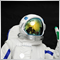2
17 263

### Introduction

In order to get a better understanding of this library's purpose, please read the first article: Graphical interfaces I: Preparation of the library structure (Chapter 1). You will find a list of articles with links at the end of each chapter. There, you can also download a complete version of the library at the current stage of development. The files must be located in the same directories as in the archive.

This article considers a new control: the Multiline Text box. Unlike the graphical objects of the OBJ_EDIT type provided in the terminal, the presented version will not have restrictions on the number of input characters. It allows to turn the text box into a simple text editor. That is, it will be possible to enter multiple lines, and the text cursor will be movable both by mouse and keys. If the lines overflow the visible area of the control, a scrollbar will appear. The Multiline Text box control will be fully rendered, and it will have a quality as close as possible to the similar control in operating systems.

### Key groups and keyboard layouts

Before describing the code of the CTextBox (Text box) type control, the keyboard should be briefly covered, as it will be the means of data input. Also, denote pressing of which keys will be handled in the first version of the control class.

The keyboard keys can be divided into several groups (see notation in Fig. 1):

• Control keys (orange)
• Function keys (purple)
• Alphanumeric keys (blue)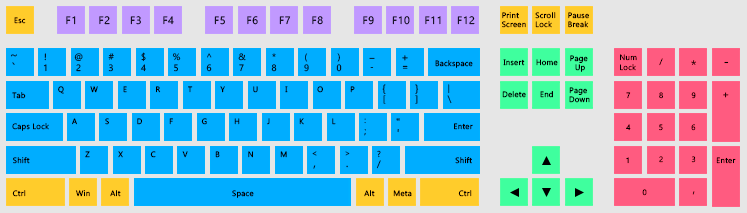Fig. 1. Key groups (QWERTY keyboard layout).

There are multiple Latin keyboard layouts for the English language. The most popular one is QWERTY. In our case, the main language is Russian, so we use the Russian language layout - ЙЦУКЕН. QWERTY is left for the English language, which is selected as the additional one.

Starting with build 1510, the MQL language includes the ::TranslateKey() function. It can be used for obtaining the character from the passed code of the pressed key, which corresponds to the language and layout set in the operating system. Previously, the arrays of characters had to be manually generated for each language, which caused difficulties due to the large amount of work. Now everything is much easier.

### Handling the keypress event

The keypress events can be tracked in the ::OnChartEvent() system function using the CHARTEVENT_KEYDOWN identifier:

```//+------------------------------------------------------------------+
//| ChartEvent function                                              |
//+------------------------------------------------------------------+
void OnChartEvent(const int id,const long &lparam,const double &dparam,const string &sparam)
{
//--- Keypress
if(id==CHARTEVENT_KEYDOWN)
{
::Print("id: ",id,"; lparam: ",lparam,"; dparam: ",dparam,"; sparam: ",sparam,"; symbol: ",::ShortToString(::TranslateKey((int)lparam)));
return;
}
}
```

The following values go to the function as the other three parameters:

• long parameter (lparam) – code of the pressed key, i.e. the ASCII code of the character or code of a control key.
• dparam parameter (dparam) – the number of keypresses generated while the key was held in the pressed state. The value is always equal to 1. In case it is necessary to get the number of call from the moment the key was being pressed, calculation is performed independently.
• sparam parameter (sparam) – string value of the bit mask, which describes the status of the keyboard keys. The event is generated immediately when a key is pressed. If the key is pressed and released right away, without holding it, the value of the scan code will be received here. If the key is pressed and held afterwards, a value will be generated based on the scan code + 16384 bits.

For example, the listing below (output in the terminal log) shows the result of pressing and holding the Esc key. The code of this key is 27(lparam), scan code at the time of pressing is 1 (sparam), and when held for approximately 500 milliseconds, the terminal begins to generate a value of 16385 (scan code + 16384 bits).

```2017.01.20 17:53:33.240 id: 0; lparam: 27; dparam: 1.0; sparam: 1
2017.01.20 17:53:33.739 id: 0; lparam: 27; dparam: 1.0; sparam: 16385
2017.01.20 17:53:33.772 id: 0; lparam: 27; dparam: 1.0; sparam: 16385
2017.01.20 17:53:33.805 id: 0; lparam: 27; dparam: 1.0; sparam: 16385
2017.01.20 17:53:33.837 id: 0; lparam: 27; dparam: 1.0; sparam: 16385
2017.01.20 17:53:33.870 id: 0; lparam: 27; dparam: 1.0; sparam: 16385
...
```

Not all keys raise events with the CHARTEVENT_KEYDOWN identifier. Some of them are allocated to the needs of the terminal, and others simply do not generate the keypress event. They are highlighted in blue in the figure below: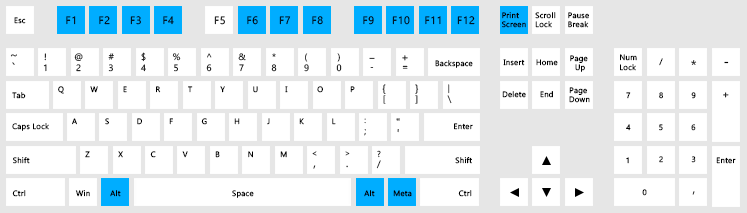Fig. 2. Keys occupied by the terminal, which do not generate the CHARTEVENT_KEYDOWN event.

### ASCII codes of characters and control keys

Information from Wikipedia (more):

ASCII, abbreviated from American Standard Code for Information Interchange, is a character encoding standard. ASCII codes represent text in computers, telecommunications equipment, and other devices. The first edition of the standard was published in 1963.

The figure below shows the ASCII codes of keyboard keys: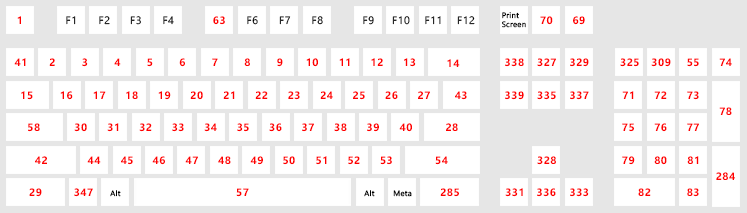Fig. 3. ASCII codes of keys.

All ASCII codes have been placed in the KeyCodes.mqh file in the form of macro substitution (#define). The listing below show a portion of these codes:

```//+------------------------------------------------------------------+
//|                                                     KeyCodes.mqh |
//|                        Copyright 2016, MetaQuotes Software Corp. |
//|                                              https://www.mql5.com |
//+------------------------------------------------------------------+
//+------------------------------------------------------------------+
//| Codes of ASCII characters and control keys                       |
//| for handling the keypress event (long parameter of the event)    |
//+------------------------------------------------------------------+
#define KEY_BACKSPACE          8
#define KEY_TAB                9
#define KEY_ENTER              13
#define KEY_SHIFT              16
#define KEY_CTRL               17
#define KEY_BREAK              19
#define KEY_CAPS_LOCK          20
#define KEY_ESC                27
#define KEY_SPACE              32
#define KEY_PAGE_UP            33
#define KEY_PAGE_DOWN          34
#define KEY_END                35
#define KEY_HOME               36
#define KEY_LEFT               37
#define KEY_UP                 38
#define KEY_RIGHT              39
#define KEY_DOWN               40
#define KEY_INSERT             45
#define KEY_DELETE             46
...
```

### Key Scan Codes

Information from Wikipedia (more):

A scancode (or scan code) is the data that most computer keyboards send to a computer to report which keys have been pressed. A number, or sequence of numbers, is assigned to each key on the keyboard.

The figure below shows the key scan codes: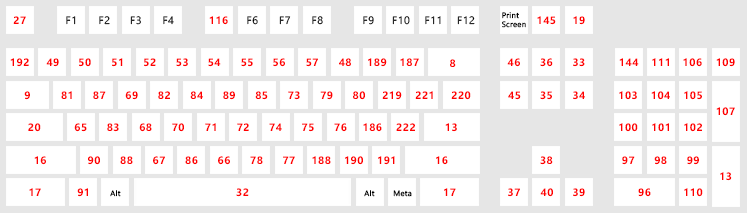Fig. 4. Key Scan Codes.

Similar to the ASCII codes, the scan codes are contained in the KeyCodes.mqh file. The listing below shows a part of the list:

```//+------------------------------------------------------------------+
//|                                                     KeyCodes.mqh |
//|                        Copyright 2016, MetaQuotes Software Corp. |
//|                                              https://www.mql5.com |
//+------------------------------------------------------------------+
...
//--- Bit
#define KEYSTATE_ON            16384
//+------------------------------------------------------------------+
//| Key Scan Codes (string parameter of the event)                   |
//+------------------------------------------------------------------+
//| Pressed once: KEYSTATE_XXX                                       |
//| Pressed down: KEYSTATE_XXX + KEYSTATE_ON                         |
//+------------------------------------------------------------------+
#define KEYSTATE_ESC           1
#define KEYSTATE_1             2
#define KEYSTATE_2             3
#define KEYSTATE_3             4
#define KEYSTATE_4             5
#define KEYSTATE_5             6
#define KEYSTATE_6             7
#define KEYSTATE_7             8
#define KEYSTATE_8             9
#define KEYSTATE_9             10
#define KEYSTATE_0             11
//---
#define KEYSTATE_MINUS         12
#define KEYSTATE_EQUALS        13
#define KEYSTATE_BACKSPACE     14
#define KEYSTATE_TAB           15
//---
#define KEYSTATE_Q             16
#define KEYSTATE_W             17
#define KEYSTATE_E             18
#define KEYSTATE_R             19
#define KEYSTATE_T             20
#define KEYSTATE_Y             21
#define KEYSTATE_U             22
#define KEYSTATE_I             23
#define KEYSTATE_O             24
#define KEYSTATE_P             25
...
```

### Auxiliary class for working with the keyboard

For more convenient work with the keyboard, the CKeys class has been implemented. It is contained in the Keys.mqh class, and includes the KeyCodes.mqh file with all key and character codes.

```//+------------------------------------------------------------------+
//|                                                         Keys.mqh |
//|                        Copyright 2016, MetaQuotes Software Corp. |
//|                                              https://www.mql5.com |
//+------------------------------------------------------------------+
#include <EasyAndFastGUI\KeyCodes.mqh>
//+------------------------------------------------------------------+
//| Class for working with the keyboard                              |
//+------------------------------------------------------------------+
class CKeys
{
public:
CKeys(void);
~CKeys(void);
};
//+------------------------------------------------------------------+
//| Constructor                                                      |
//+------------------------------------------------------------------+
CKeys::CKeys(void)
{
}
//+------------------------------------------------------------------+
//| Destructor                                                       |
//+------------------------------------------------------------------+
CKeys::~CKeys(void)
{
}
```

To determine a keypress with:

(1) alphanumeric character (including Space)

or (3) special character,

use the CKeys::KeySymbol() method. If it is passed a value of the long parameter of the event with the CHARTEVENT_KEYDOWN identifier, it will return a character in string format or an empty string (''), in case the pressed key does not belong to the specified ranges.

```class CKeys
{
public:
//--- Returns the character of the pressed key
string            KeySymbol(const long key_code);
};
//+------------------------------------------------------------------+
//| Returns the character of the pressed key                         |
//+------------------------------------------------------------------+
string CKeys::KeySymbol(const long key_code)
{
string key_symbol="";
//--- If it is necessary to enter a space (Space key)
if(key_code==KEY_SPACE)
{
key_symbol=" ";
}
//--- If it is necessary to enter (1) an alphabetic character, or (2) a numeric pad character, or (3) a special character
else if((key_code>=KEY_A && key_code<=KEY_Z) ||
(key_code>=KEY_0 && key_code<=KEY_9) ||
(key_code>=KEY_SEMICOLON && key_code<=KEY_SINGLE_QUOTE))
{
key_symbol=::ShortToString(::TranslateKey((int)key_code));
}
//--- Return the character
return(key_symbol);
}
```

And finally, a method will be required to determine the current state of the Ctrl key. It will be used in various combinations of simultaneously pressing two keys when moving the text cursor in the text box.

To get the current state of the Ctrl key, use the terminal's system function ::TerminalInfoInteger(). This function has multiple identifiers for detecting the current state of the keys. The TERMINAL_KEYSTATE_CONTROL identifier is intended for the Ctrl key. All other identifiers of this type can be found in the MQL5 language reference.

It is very easy to determine if a key is pressed using the identifiers. If a key is pressed, the return value will be less than zero

```class CKeys
{
public:
//--- Returns the state of the Ctrl key
bool              KeyCtrlState(void);
};
//+------------------------------------------------------------------+
//| Returns the state of the Ctrl key                                |
//+------------------------------------------------------------------+
bool CKeys::KeyCtrlState(void)
{
return(::TerminalInfoInteger(TERMINAL_KEYSTATE_CONTROL)<0);
}
```

Now, everything is ready for the creating the Text box control.

### The Multiline Text box control

The Multiline Text box control can be also used in combined controls. It belongs to the group of compound controls, as it contains scrollbars. Additionally, the Multiline Text box control can be used for both entering text and displaying the previously saved text from a file.

The controls with edit boxes for entering numeric values (the CSpinEdit class) or custom text (CTextEdit) have been considered earlier. They used a graphical object of the OBJ_EDIT type. It has a serious limitation: only 63 characters can be entered, and they must fit in a single line too. Therefore, the current task is to create a text edit box without such limitations.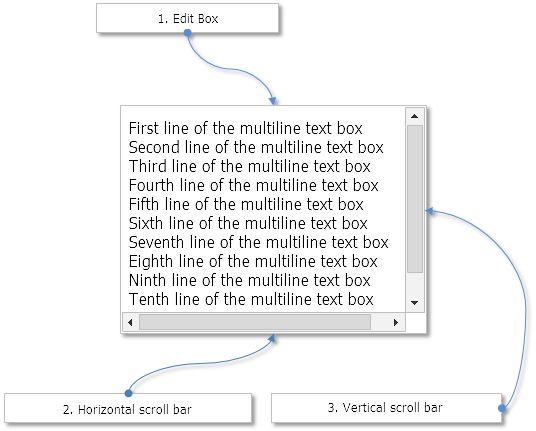Fig. 5. The Multiline Text box control.

Now let us take a closer look at the CTextBox class for creating this control.

### Developing the CTextBox class for creating the control

Create the TextBox.mqh file with the CTextBox class which has methods standard for all controls of the library and include the following files in it:

• With the base class of controls — Element.mqh.
• With the scrollbar classes — Scrolls.mqh.
• With the class for working with the keyboard — Keys.mqh.
• With the class for working with the time counter — TimeCounter.mqh.
• With the class for working with the chart, where the MQL is located — Chart.mqh

```//+------------------------------------------------------------------+
//|                                                      TextBox.mqh |
//|                        Copyright 2016, MetaQuotes Software Corp. |
//|                                              https://www.mql5.com |
//+------------------------------------------------------------------+
#include "Scrolls.mqh"
#include "..\Keys.mqh"
#include "..\Element.mqh"
#include "..\TimeCounter.mqh"
#include <Charts\Chart.mqh>
//+------------------------------------------------------------------+
//| Class for creating a multiline text box                          |
//+------------------------------------------------------------------+
class CTextBox : public CElement
{
private:
//--- Instance of the class for working with the keyboard
CKeys             m_keys;
//--- Class instance for managing the chart
CChart            m_chart;
//--- Instance of the class for working with the time counter
CTimeCounter      m_counter;
//---
public:
CTextBox(void);
~CTextBox(void);
//--- Chart event handler
virtual void      OnEvent(const int id,const long &lparam,const double &dparam,const string &sparam);
//--- Timer
virtual void      OnEventTimer(void);
//--- Moving the element
virtual void      Moving(const int x,const int y,const bool moving_mode=false);
//--- (1) Show, (2) hide, (3) reset, (4) delete
virtual void      Show(void);
virtual void      Hide(void);
virtual void      Reset(void);
virtual void      Delete(void);
//--- (1) Set, (2) reset priorities of the left mouse button click
virtual void      SetZorders(void);
virtual void      ResetZorders(void);
//--- Zero the color
virtual void      ResetColors(void) {}
//---
private:
//--- Change the width at the right edge of the window
virtual void      ChangeWidthByRightWindowSide(void);
//--- Change the height at the bottom edge of the window
virtual void      ChangeHeightByBottomWindowSide(void);
};
```

### Properties and appearance

A structure will be required, name it KeySymbolOptions, with arrays of characters and their properties. In the current version, it will contain two dynamic arrays:

• The m_symbol[] will contain all characters of a string, separately.
• The m_width[] array will contain the width of all characters of the string, separately.

An instance of this class will also be declared as a dynamic array. Its size will always be equal to the number of lines in the text box.

```class CTextBox : public CElement
{
private:
//--- Characters and their properties
struct KeySymbolOptions
{
string            m_symbol[]; // Characters
int               m_width[];  // Width of the characters
};
KeySymbolOptions  m_lines[];
};
```

In the first version of the control, the text will be output as entire lines. Therefore, before a line is output, it needs to be put together from the m_symbol[] array. The CTextBox::CollectString() method serves this purpose, which needs to be passed the line index:

```class CTextBox : public CElement
{
private:
//--- Variable for working with a string
string            m_temp_input_string;
//---
private:
//--- Builds a string from characters
string            CollectString(const uint line_index);
};
//+------------------------------------------------------------------+
//| Builds a string from characters                                  |
//+------------------------------------------------------------------+
string CTextBox::CollectString(const uint line_index)
{
m_temp_input_string="";
uint symbols_total=::ArraySize(m_lines[line_index].m_symbol);
for(uint i=0; i<symbols_total; i++)
//---
return(m_temp_input_string);
}
```

Next, enumerate the properties of the text edit box, which can be used to customize the appearance of this control, as well as its state and the modes it can work in:

• Color of the background in different states
• Color of the text in different states
• Color of the frame in different states
• Default text
• Default text color
• Multiline mode

```class CTextBox : public CElement
{
private:
//--- Background color
color             m_area_color;
color             m_area_color_locked;
//--- Text color
color             m_text_color;
color             m_text_color_locked;
//--- Frame color
color             m_border_color;
color             m_border_color_hover;
color             m_border_color_locked;
color             m_border_color_activated;
//--- Default text
string            m_default_text;
//--- Default text color
color             m_default_text_color;
//--- Multiline mode
bool              m_multi_line_mode;
//---
public:
//--- Color of the background in different states
void              AreaColor(const color clr)                { m_area_color=clr;                 }
void              AreaColorLocked(const color clr)          { m_area_color_locked=clr;          }
//--- Color of the text in different states
void              TextColor(const color clr)                { m_text_color=clr;                 }
void              TextColorLocked(const color clr)          { m_text_color_locked=clr;          }
//--- Colors of the frame in different states
void              BorderColor(const color clr)              { m_border_color=clr;               }
void              BorderColorHover(const color clr)         { m_border_color_hover=clr;         }
void              BorderColorLocked(const color clr)        { m_border_color_locked=clr;        }
void              BorderColorActivated(const color clr)     { m_border_color_activated=clr;     }
//--- (1) Default text and (2) default text color
void              DefaultText(const string text)            { m_default_text=text;              }
void              DefaultTextColor(const color clr)         { m_default_text_color=clr;         }
//--- (1) Multiline mode, (2) read-only mode
void              MultiLineMode(const bool mode)            { m_multi_line_mode=mode;           }
};
```

The text box itself (background, text, frame and blinking text cursor) will be completely drawn on a single graphical object of the OBJ_BITMAP_LABEL type. In essence, this is just a picture. It will be redrawn in two cases:

• when interacting with the control
• at a specified time interval, for the cursor to blink when the text box is activated.

When the mouse cursor hovers the text box area, its frame will change color. In order to avoid redrawing the picture too frequently, it is necessary to track the moment when the cursor crosses the text box frame. That is, the control should be redrawn only once, the moment the cursor enters or exits the text box area. For these purposes, the CElementBase::IsMouseFocus() methods have been added to the base class of the control. They are used to set and get the flag, which indicates a crossing:

```//+------------------------------------------------------------------+
//| Base control class                                               |
//+------------------------------------------------------------------+
class CElementBase
{
protected:
//--- To determine the moment when the mouse cursor crosses the borders of the control
bool              m_is_mouse_focus;
//---
public:
//--- The moment of entering/exiting the focus of the control
bool              IsMouseFocus(void)                        const { return(m_is_mouse_focus);             }
void              IsMouseFocus(const bool focus)                  { m_is_mouse_focus=focus;               }
};
```

For the code to be simple and readable, additional simple methods have been implemented within it, which help to obtain the color of the text box background, frame and text relative to the current state of the control:

```class CTextBox : public CElement
{
private:
//--- Returns the current background color
uint              AreaColorCurrent(void);
//--- Returns the current text color
uint              TextColorCurrent(void);
//--- Returns the current frame color
uint              BorderColorCurrent(void);
};
//+------------------------------------------------------------------+
//| Returns background color relative to current state of control    |
//+------------------------------------------------------------------+
uint CTextBox::AreaColorCurrent(void)
{
uint clr=::ColorToARGB((m_text_box_state)? m_area_color : m_area_color_locked);
//--- Return the color
return(clr);
}
//+------------------------------------------------------------------+
//| Returns text color relative to current state of control          |
//+------------------------------------------------------------------+
uint CTextBox::TextColorCurrent(void)
{
uint clr=::ColorToARGB((m_text_box_state)? m_text_color : m_text_color_locked);
//--- Return the color
return(clr);
}
//+------------------------------------------------------------------+
//| Returns frame color relative to current state of control         |
//+------------------------------------------------------------------+
uint CTextBox::BorderColorCurrent(void)
{
uint clr=clrBlack;
//--- If the element is not blocked
if(m_text_box_state)
{
//--- If the text box is activated
if(m_text_edit_state)
clr=m_border_color_activated;
//--- If not activated, check the control focus
else
clr=(CElementBase::IsMouseFocus())? m_border_color_hover : m_border_color;
}
//--- If the control is blocked
else
clr=m_border_color_locked;
//--- Return the color
return(::ColorToARGB(clr));
}
```

In many methods of the class, it will be necessary to obtain the value of text box line height in pixels relative to the specified font and its size. For these purposes, use the CTextBox::LineHeight() method:

```class CTextBox : public CElement
{
private:
//--- Returns the line height
uint              LineHeight(void);
};
//+------------------------------------------------------------------+
//| Returns the line height                                          |
//+------------------------------------------------------------------+
uint CTextBox::LineHeight(void)
{
//--- Set the font to be displayed on the canvas (required for getting the line height)
m_canvas.FontSet(CElementBase::Font(),-CElementBase::FontSize()*10,FW_NORMAL);
//--- Return the line height
return(m_canvas.TextHeight("|"));
}
```

Now consider the methods for drawing the control. Start with the CTextBox::DrawBorder() method designed for drawing the text box border. If the total size of the text box is greater than its visible part, the visibility area can be offset (using the scrollbars or cursor). Therefore, the frame should be drawn with consideration of these offsets

```class CTextBox : public CElement
{
private:
//--- Draws the frame
void              DrawBorder(void);
};
//+------------------------------------------------------------------+
//| Draws the frame of the Text box                                  |
//+------------------------------------------------------------------+
void CTextBox::DrawBorder(void)
{
//--- Get the frame color relative to current state of control
uint clr=BorderColorCurrent();
//--- Get the offset along the X axis
int xo=(int)m_canvas.GetInteger(OBJPROP_XOFFSET);
int yo=(int)m_canvas.GetInteger(OBJPROP_YOFFSET);
//--- Boundaries
int x_size =m_canvas.X_Size()-1;
int y_size =m_canvas.Y_Size()-1;
//--- Coordinates: top/right/bottom/left
int x1; x1=x;         x1=x_size+xo; x1=xo;        x1=x;
int y1; y1=y;         y1=y;         y1=y_size+yo; y1=y;
int x2; x2=x_size+xo; x2=x_size+xo; x2=x_size+xo; x2=x;
int y2; y2=y;         y2=y_size+yo; y2=y_size+yo; y2=y_size+yo;
//--- Draw the frame by specified coordinates
for(int i=0; i<4; i++)
m_canvas.Line(x1[i],y1[i],x2[i],y2[i],clr);
}
```

The CTextBox::DrawBorder() method will also be used in the CTextBox::ChangeObjectsColor() method, when it is necessary to simply change the frame color of the text box when it is hovered by mouse cursor (see the code below). To do this, simply redraw the frame (and not the entire text box) and refresh the image. The CTextBox::ChangeObjectsColor() will be called within the event handler of the control. This is where the act of the mouse cursor crossing the control borders is tracked in order avoid too frequent redraws.

```class CTextBox : public CElement
{
private:
//--- Changing the object colors
void              ChangeObjectsColor(void);
};
//+------------------------------------------------------------------+
//| Changing the object colors                                       |
//+------------------------------------------------------------------+
void CTextBox::ChangeObjectsColor(void)
{
//--- If not in focus
if(!CElementBase::MouseFocus())
{
//--- If not yet indicated that not in focus
if(CElementBase::IsMouseFocus())
{
//--- Set the flag
CElementBase::IsMouseFocus(false);
//--- Change the color
DrawBorder();
m_canvas.Update();
}
}
else
{
//--- If not yet indicated that in focus
if(!CElementBase::IsMouseFocus())
{
//--- Set the flag
CElementBase::IsMouseFocus(true);
//--- Change the color
DrawBorder();
m_canvas.Update();
}
}
}
```

The CTextBox::TextOut() method is designed to output text to a canvas. Here, at the very beginning, the canvas is cleared by filling with the specified color. Next, the program can go two ways:

• If the multiline mode is disabled, and at the same there is no character in the line, the default text should be displayed (if specified). It will be displayed in the center of the edit box.
• If the multiline mode is disabled or if the line contains at least one character, get the height of the line and display all lines in a loop, building them from the array of characters first. The text offsets from the top left corner of the text box area are defined by default. Those are 5 pixels along the X axis, and 4 pixels along the Y axis. These values can be overridden using the CTextBox::TextXOffset() and CTextBox::TextYOffset() methods.

```class CTextBox : public CElement
{
private:
//--- Text offsets from the text box edges
int               m_text_x_offset;
int               m_text_y_offset;
//---
public:
//--- Text offsets from the text box edges
void              TextXOffset(const int x_offset)           { m_text_x_offset=x_offset;         }
void              TextYOffset(const int y_offset)           { m_text_y_offset=y_offset;         }
//---
private:
//--- Output text to canvas
void              TextOut(void);
};
//+------------------------------------------------------------------+
//| Constructor                                                      |
//+------------------------------------------------------------------+
CTextBox::CTextBox(void) : m_text_x_offset(5),
m_text_y_offset(4)
{
...
}
//+------------------------------------------------------------------+
//| Output text to canvas                                            |
//+------------------------------------------------------------------+
void CTextBox::TextOut(void)
{
//--- Clear canvas
m_canvas.Erase(AreaColorCurrent());
//--- Get the size of the array of characters
uint symbols_total=::ArraySize(m_lines[m_text_cursor_y_pos].m_symbol);
//--- If multiline mode is enabled or if the number of characters is greater than zero
if(m_multi_line_mode || symbols_total>0)
{
//--- Get the line height
int line_height=(int)LineHeight();
//--- Get the size of the lines array
uint lines_total=::ArraySize(m_lines);
//---
for(uint i=0; i<lines_total; i++)
{
//--- Get the coordinates for the text
int x=m_text_x_offset;
int y=m_text_y_offset+((int)i*line_height);
//--- Build a string from the array of characters
CollectString(i);
//--- Draw text
m_canvas.TextOut(x,y,m_temp_input_string,TextColorCurrent(),TA_LEFT);
}
}
//--- If the multiline mode is disabled and there is no character, the default text will be displayed
else
{
//--- Draw text, if specified
if(m_default_text!="")
m_canvas.TextOut(m_area_x_size/2,m_area_y_size/2,m_default_text,::ColorToARGB(m_default_text_color),TA_CENTER|TA_VCENTER);
}
}
```

To draw the text cursor, methods for calculation of its coordinates will be required. To calculate the X coordinate, it is necessary to specify the index of the line and index of the character where the cursor is to be placed. This is done by using the CTextBox::LineWidth() method: Since the width of each character is stored in the m_width[] dynamic array of the KeySymbolOptions structure, it only remains to sum up the width of characters up to the specified position

```class CTextBox : public CElement
{
private:
//--- Returns the line width in pixels
uint              LineWidth(const uint line_index,const uint symbol_index);
};
//+------------------------------------------------------------------+
//| Returns line width from beginning to the specified position      |
//+------------------------------------------------------------------+
uint CTextBox::LineWidth(const uint line_index,const uint symbol_index)
{
//--- Get the size of the lines array
uint lines_total=::ArraySize(m_lines);
//--- Prevention of exceeding the array size
uint l=(line_index<lines_total)? line_index : lines_total-1;
//--- Get the size of the array of characters for the specified line
uint symbols_total=::ArraySize(m_lines[l].m_width);
//--- Prevention of exceeding the array size
uint s=(symbol_index<symbols_total)? symbol_index : symbols_total;
//--- Sum the width of all characters
uint width=0;
for(uint i=0; i<s; i++)
width+=m_lines[l].m_width[i];
//--- Return the line width
return(width);
}
```

Methods for obtaining the text cursor coordinates take a very simple form (see the code below). Coordinates are stored in the m_text_cursor_x and m_text_cursor_y fields. The calculation of the coordinates uses the current position of the cursor, as well as the indexes of the line and character where the cursor is to be moved. The m_text_cursor_x_pos and m_text_cursor_y_pos fields are meant for storing these values.

```class CTextBox : public CElement
{
private:
//--- Current coordinates of the text cursor
int               m_text_cursor_x;
int               m_text_cursor_y;
//--- Current position of the text cursor
uint              m_text_cursor_x_pos;
uint              m_text_cursor_y_pos;
//---
private:
//--- Calculation of coordinates for the text cursor
void              CalculateTextCursorX(void);
void              CalculateTextCursorY(void);
};
//+------------------------------------------------------------------+
//| Calculation of the X coordinate for the text cursor              |
//+------------------------------------------------------------------+
void CTextBox::CalculateTextCursorX(void)
{
//--- Get the line width
int line_width=(int)LineWidth(m_text_cursor_x_pos,m_text_cursor_y_pos);
//--- Calculate and store the X coordinate of the cursor
m_text_cursor_x=m_text_x_offset+line_width;
}
//+------------------------------------------------------------------+
//| Calculation of the Y coordinate for the text cursor              |
//+------------------------------------------------------------------+
void CTextBox::CalculateTextCursorY(void)
{
//--- Get the line height
int line_height=(int)LineHeight();
//--- Get the Y coordinate of the cursor
m_text_cursor_y=m_text_y_offset+int(line_height*m_text_cursor_y_pos);
}
```

Everything is ready for implementation of the CTextBox::DrawCursor() method for drawing the text cursor. In many other text editors, it can be noticed that the text cursor partially overlaps the pixels of some characters. It can be seen that the text cursor does not simply obstruct them. The covered pixels of the character are drawn in a different color. This is done to maintain readability of the character.

For example, the screenshot below shows the 'd' and 'д' characters in a text editor overlapped and not overlapped by the cursor.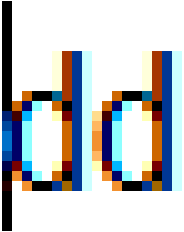Fig. 6. Example of text cursor overlapping the pixels of the 'd' character.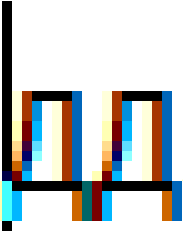Fig. 7. Example of text cursor overlapping the pixels of the 'д' character.

For the cursor and the overlapped character to visible on a background of any color at all times, it is sufficient to invert the colors of the pixels overlapped by the cursor.

Now, consider the CTextBox::DrawCursor() method for drawing the text cursor. The cursor width will be equal to one pixel, and its height will match the line height. At the very beginning, get the X coordinate at which to draw the cursor, and the line height. The Y coordinate will be calculated in a loop, as it will be drawn on a per-pixel basis. Remember, an instance of the CColors class for working with color has been previously declared in the CElementBase base class of controls. Therefore, the color of the current pixel at the specified coordinates is now obtained at each iteration after calculation of the Y coordinate. Then, the CColors::Negative() method inverts the color and sets it to the same place

```class CTextBox : public CElement
{
private:
//--- Draws the text cursor
void              DrawCursor(void);
};
//+------------------------------------------------------------------+
//| Draws the text cursor                                            |
//+------------------------------------------------------------------+
void CTextBox::DrawCursor(void)
{
//--- Get the line height
int line_height=(int)LineHeight();
//--- Get the X coordinate of the cursor
CalculateTextCursorX();
//--- Draw the text cursor
for(int i=0; i<line_height; i++)
{
//--- Get the Y coordinate of the pixel
int y=m_text_y_offset+((int)m_text_cursor_y_pos*line_height)+i;
//--- Get the current color of the pixel
uint pixel_color=m_canvas.PixelGet(m_text_cursor_x,y);
//--- Invert color for the cursor
pixel_color=m_clr.Negative((color)pixel_color);
m_canvas.PixelSet(m_text_cursor_x,y,::ColorToARGB(pixel_color));
}
}
```

Two methods have been implemented for drawing the text box with text: CTextBox::DrawText() and CTextBox::DrawTextAndCursor().

The CTextBox::DrawText() method is to be used when it is only necessary to update the text in an inactive text box. Everything is simple here. If the control is not hidden, display the text, draw the frame and update the picture.

```class CTextBox : public CElement
{
private:
//--- Draw text
void              DrawText(void);
};
//+------------------------------------------------------------------+
//| Draws the text                                                   |
//+------------------------------------------------------------------+
void CTextBox::DrawText(void)
{
//--- Leave, if the control is hidden
if(!CElementBase::IsVisible())
return;
//--- Output the text
CTextBox::TextOut();
//--- Draw the frame
DrawBorder();
//--- Update the text box
m_canvas.Update();
}
```

If the text box is active, in addition to text, it is necessary to display a blinking text cursor - the CTextBox::DrawTextAndCursor() method. For blinking it is necessary to determine the state to show/hide the cursor. Every time this method is called, the state will be changed to the opposite. It also provided the ability to force the display when the true value (the show_state argument) is passed to the method. The forced display will be required when moving the cursor in the text box while it is active. In fact, the blinking of the cursor will be carried out in a timer of the control at the interval specified in the class constructor of the time counter. Here, its value is 200 milliseconds. The counter must be reset every time after calling the CTextBox::DrawTextAndCursor() method.

```class CTextBox : public CElement
{
private:
//--- Displays the text and blinking cursor
void              DrawTextAndCursor(const bool show_state=false);
};
//+------------------------------------------------------------------+
//| Constructor                                                      |
//+------------------------------------------------------------------+
CTextBox::CTextBox(void)
{
//--- Setting parameters for the timer counter
m_counter.SetParameters(16,200);
}
//+------------------------------------------------------------------+
//| Timer                                                            |
//+------------------------------------------------------------------+
void CTextBox::OnEventTimer(void)
{
...
//--- Pause between updates of the text cursor
if(m_counter.CheckTimeCounter())
{
//--- Update the text cursor if the control is visible and the text box is activated
if(CElementBase::IsVisible() && m_text_edit_state)
DrawTextAndCursor();
}
}
//+------------------------------------------------------------------+
//| Displays the text and blinking cursor                            |
//+------------------------------------------------------------------+
void CTextBox::DrawTextAndCursor(const bool show_state=false)
{
//--- Determine the state for the text cursor (show/hide)
static bool state=false;
state=(!show_state)? !state : show_state;
//--- Output the text
CTextBox::TextOut();
//--- Draw the text cursor
if(state)
DrawCursor();
//--- Draw the frame
DrawBorder();
//--- Update the text box
m_canvas.Update();
//--- Reset the counter
m_counter.ZeroTimeCounter();
}
```

To create the Multiline Text box control, three private methods, two of which are needed to create scrollbars, and one public method for external calls in a custom class will be required:

```class CTextBox : public CElement
{
private:
//--- Objects for creating the element
CRectCanvas       m_canvas;
CScrollV          m_scrollv;
CScrollH          m_scrollh;
//---
public:
//--- Methods for creating the control
bool              CreateTextBox(const long chart_id,const int subwin,const int x_gap,const int y_gap);
//---
private:
bool              CreateCanvas(void);
bool              CreateScrollV(void);
bool              CreateScrollH(void);
//---
public:
//--- Returns pointers to the scrollbars
CScrollV         *GetScrollVPointer(void)                   { return(::GetPointer(m_scrollv));  }
CScrollH         *GetScrollHPointer(void)                   { return(::GetPointer(m_scrollh));  }
};
```

Before calling the CTextBox::CreateCanvas() method to create the text box, it is necessary to calculate its size. A method similar to the one implemented in the rendered table of the CCanvasTable type will be applied here. Let us go over it briefly. There is the total size of the image, and there is the size of its visible part. The size of a control is equal to the size of the image's visible part. When moving the text cursor or scrollbars, coordinates of the image will change, while the coordinates of the visible part (which are also the control coordinates) will remain the same.

The size along the Y axis can be calculated by simply multiplying the number of lines by their height. The margins from the text box edges and the size of the scrollbar are also considered here. To calculate the size along the X axis, it is necessary to know the maximum line width of the entire array. This is done by using the CTextBox::MaxLineWidth() method. Here, it iterates over the lines array in a cycle, stores the full width of the line if it is greater than the previous one, and returns the value.

```class CTextBox : public CElement
{
private:
//--- Returns the maximum line width
uint              MaxLineWidth(void);
};
//+------------------------------------------------------------------+
//| Returns the maximum line width                                   |
//+------------------------------------------------------------------+
uint CTextBox::MaxLineWidth(void)
{
uint max_line_width=0;
//--- Get the size of the lines array
uint lines_total=::ArraySize(m_lines);
for(uint i=0; i<lines_total; i++)
{
//--- Get the size of the array of characters
uint symbols_total=::ArraySize(m_lines[i].m_symbol);
//--- Get the line width
uint line_width=LineWidth(symbols_total,i);
//--- Store the maximum width
if(line_width>max_line_width)
max_line_width=line_width;
}
//--- Return the maximum line width
return(max_line_width);
}
```

The code of the CTextBox::CalculateTextBoxSize() method for calculating the control sizes is as shown in the listing below. This method will also be called from within the CTextBox::ChangeWidthByRightWindowSide() and CTextBox::ChangeHeightByBottomWindowSide() methods. The purpose of those methods is to automatically resize the control according to the form size, if such properties are defined by the developer.

```class CTextBox : public CElement
{
private:
//--- Total size and size of the visible part of the control
int               m_area_x_size;
int               m_area_y_size;
int               m_area_visible_x_size;
int               m_area_visible_y_size;
//---
private:
//--- Calculates the width of the text box
void              CalculateTextBoxSize(void);
};
//+------------------------------------------------------------------+
//| Calculates the size of the text box                              |
//+------------------------------------------------------------------+
void CTextBox::CalculateTextBoxSize(void)
{
//--- Get the maximum line width from the text box
int max_line_width=int((m_text_x_offset*2)+MaxLineWidth()+m_scrollv.ScrollWidth());
//--- Determine the total width
m_area_x_size=(max_line_width>m_x_size)? max_line_width : m_x_size;
//--- Determine the visible width
m_area_visible_x_size=m_x_size;
//--- Get the line height
int line_height=(int)LineHeight();
//--- Get the size of the lines array
int lines_total=::ArraySize(m_lines);
//--- Calculate the total height of the control
int lines_height=int((m_text_y_offset*2)+(line_height*lines_total)+m_scrollh.ScrollWidth());
//--- Determine the total height
m_area_y_size=(m_multi_line_mode && lines_height>m_y_size)? lines_height : m_y_size;
//--- Determine the visible height
m_area_visible_y_size=m_y_size;
}
```

The sizes have been calculated. Now they need to be applied. This is done by using the CTextBox::ChangeTextBoxSize() method. The method arguments here specify if it is necessary to shift the visibility area to the beginning or to leave it at the same position. In addition, this method resizes the scrollbars and performs the final adjustment of the visibility area relative to the scrollbar thumbs. The code of those methods will not be covered here, because a similar case has already been described in previous articles.

```class CTextBox : public CElement
{
private:
//--- Resize the text box
void              ChangeTextBoxSize(const bool x_offset=false,const bool y_offset=false);
};
//+------------------------------------------------------------------+
//| Resize the text box                                              |
//+------------------------------------------------------------------+
void CTextBox::ChangeTextBoxSize(const bool is_x_offset=false,const bool is_y_offset=false)
{
//--- Resize the table
m_canvas.XSize(m_area_x_size);
m_canvas.YSize(m_area_y_size);
m_canvas.Resize(m_area_x_size,m_area_y_size);
//--- Set the size of the visible area
m_canvas.SetInteger(OBJPROP_XSIZE,m_area_visible_x_size);
m_canvas.SetInteger(OBJPROP_YSIZE,m_area_visible_y_size);
//--- Difference between the total width and visible area
int x_different=m_area_x_size-m_area_visible_x_size;
int y_different=m_area_y_size-m_area_visible_y_size;
//--- Set the frame offset within the image along the X and Y axes
int x_offset=(int)m_canvas.GetInteger(OBJPROP_XOFFSET);
int y_offset=(int)m_canvas.GetInteger(OBJPROP_YOFFSET);
m_canvas.SetInteger(OBJPROP_XOFFSET,(!is_x_offset)? 0 : (x_offset<=x_different)? x_offset : x_different);
m_canvas.SetInteger(OBJPROP_YOFFSET,(!is_y_offset)? 0 : (y_offset<=y_different)? y_offset : y_different);
//--- Resize the scrollbars
ChangeScrollsSize();
ShiftData();
}
```

The following fields and methods are intended for managing the state of the control and for getting its current state:

• The CTextBox::TextEditState() method retrieves the state of the control.
• Calling the CTextBox::TextBoxState() method blocks/unblocks the control. A blocked control is transferred to the Read-only mode. The corresponding colors will be set to the background, frame and text (this can be done by user before creating the control).

```class CTextBox : public CElement
{
private:
//--- State of the text edit box
bool              m_text_edit_state;
//--- Control state
bool              m_text_box_state;
//---
public:
//--- (1) State of the text edit box, (2) get/set the availability state of the control
bool              TextEditState(void)                 const { return(m_text_edit_state);        }
bool              TextBoxState(void)                  const { return(m_text_box_state);         }
void              TextBoxState(const bool state);
};
//+------------------------------------------------------------------+
//| Setting the availability state of the control                    |
//+------------------------------------------------------------------+
void CTextBox::TextBoxState(const bool state)
{
m_text_box_state=state;
//--- Setting in relation to the current state
if(!m_text_box_state)
{
//--- Priorities
m_canvas.Z_Order(-1);
//--- The edit box in the Read-only mode
}
else
{
//--- Priorities
m_canvas.Z_Order(m_text_edit_zorder);
//--- The edit control in the edit mode
}
//--- Update the text box
DrawText();
}
```

### Managing the text cursor

The text edit box is activated when it is clicked. The coordinates of the clicked place are determined immediately, and the text cursor is moved there. This is done by the CTextBox::OnClickTextBox() method. But before moving on to its description, first consider some auxiliary methods that are invoked in it, as well as in many other methods of the CTextBox class.

The CTextBox::SetTextCursor() method for updating the values of the text cursor position. In the single-line mode the position along the Y axis is always equal to 0.

```class CTextBox : public CElement
{
private:
//--- Current position of the text cursor
uint              m_text_cursor_x_pos;
uint              m_text_cursor_y_pos;
//---
private:
//--- Set the cursor at the specified position
void              SetTextCursor(const uint x_pos,const uint y_pos);
};
//+------------------------------------------------------------------+
//| Set the cursor at the specified position                         |
//+------------------------------------------------------------------+
void CTextBox::SetTextCursor(const uint x_pos,const uint y_pos)
{
m_text_cursor_x_pos=x_pos;
m_text_cursor_y_pos=(!m_multi_line_mode)? 0 : y_pos;
}
```

Methods for controlling the scrollbars. Similar methods have already been covered in the previous article of the series, therefore the code will not be shown here. A brief reminder: if a parameter is not passed, the thumb will be moved to the last position, that is, to the end of the list/text/document.

```class CTextBox : public CElement
{
public:
//--- Table scrolling: (1) vertical and (2) horizontal
void              VerticalScrolling(const int pos=WRONG_VALUE);
void              HorizontalScrolling(const int pos=WRONG_VALUE);
};
```

The CTextBox::DeactivateTextBox() is required for deactivating the text box. A new feature provided by the developers of the terminal should be mentioned here. One more chart identifier (CHART_KEYBOARD_CONTROL) has been added to the ENUM_CHART_PROPERTY enumeration. It enables or disables managing the chart using the 'Left', 'Right', 'Home', 'End', 'Page Up', 'Page Down' keys, as well as chart zooming keys - '+' and '-'. Thus, when the text box is activated, it is necessary to disable chart management feature, so that the listed keys are not intercepted and this, in its turn, does not interrupt operation of the text box. When the text box is deactivated, it is necessary to re-enable managing the chart using a keyboard.

Here, it is necessary to redraw the text box, and if it is not the multiline mode, move the text cursor and the scrollbar thumb to the beginning of the line.

```class CTextBox : public CElement
{
private:
//--- Deactivates the text box
void              DeactivateTextBox(void);
};
//+------------------------------------------------------------------+
//| Deactivation of the text box                                     |
//+------------------------------------------------------------------+
void CTextBox::DeactivateTextBox(void)
{
//--- Leave, if it is already deactivated
if(!m_text_edit_state)
return;
//--- Deactivate
m_text_edit_state=false;
//--- Enable chart management
m_chart.SetInteger(CHART_KEYBOARD_CONTROL,true);
//--- Draw text
DrawText();
//--- If the multiline mode is disabled
if(!m_multi_line_mode)
{
//--- Move the cursor to the beginning of the line
SetTextCursor(0,0);
//--- Move the scrollbar to the beginning of the line
HorizontalScrolling(0);
}
}
```

When managing the text cursor, it is necessary to keep track of whether it has crossed the boundaries of the visibility area. If an intersection had occurred, the cursor must be returned to the visibility area again. For this purpose, additional reusable methods are required. The allowed boundaries of the text box must be calculated, taking into account the multiline mode and presence of scrollbars.

In order to calculate how much the visibility area is to be shifted, the current offset value must be found first:

```class CTextBox : public CElement
{
private:
//--- For calculation of the boundaries of the visible area of the text box
int               m_x_limit;
int               m_y_limit;
int               m_x2_limit;
int               m_y2_limit;
//---
private:
//--- Calculation of the text box boundaries
void              CalculateBoundaries(void);
void              CalculateXBoundaries(void);
void              CalculateYBoundaries(void);
};
//+------------------------------------------------------------------+
//| Calculation of the text box boundaries along the two axes        |
//+------------------------------------------------------------------+
void CTextBox::CalculateBoundaries(void)
{
CalculateXBoundaries();
CalculateYBoundaries();
}
//+------------------------------------------------------------------+
//| Calculation of the text box boundaries along the X axis          |
//+------------------------------------------------------------------+
void CTextBox::CalculateXBoundaries(void)
{
//--- Get the X coordinate and offset along the X axis
int x       =(int)m_canvas.GetInteger(OBJPROP_XDISTANCE);
int xoffset =(int)m_canvas.GetInteger(OBJPROP_XOFFSET);
//--- Calculate the boundaries of the visible portion of the text box
m_x_limit  =(x+xoffset)-x;
m_x2_limit =(m_multi_line_mode)? (x+xoffset+m_x_size-m_scrollv.ScrollWidth()-m_text_x_offset)-x : (x+xoffset+m_x_size-m_text_x_offset)-x;
}
//+------------------------------------------------------------------+
//| Calculation of the text box boundaries along the Y axis          |
//+------------------------------------------------------------------+
void CTextBox::CalculateYBoundaries(void)
{
//--- Leave, if the multiline mode is disabled
if(!m_multi_line_mode)
return;
//--- Get the Y coordinate and offset along the Y axis
int y       =(int)m_canvas.GetInteger(OBJPROP_YDISTANCE);
int yoffset =(int)m_canvas.GetInteger(OBJPROP_YOFFSET);
//--- Calculate the boundaries of the visible portion of the text box
m_y_limit  =(y+yoffset)-y;
m_y2_limit =(y+yoffset+m_y_size-m_scrollh.ScrollWidth())-y;
}
```

To accurately position the scrollbars relative to the current cursor position, the following methods will be used:

```class CTextBox : public CElement
{
private:
//--- Calculation of the X position of the scrollbar thumb on the left edge of the text box
int               CalculateScrollThumbX(void);
//--- Calculation of the X position of the scrollbar thumb on the right edge of the text box
int               CalculateScrollThumbX2(void);
//--- Calculation of the Y position of the scrollbar thumb on the top edge of the text box
int               CalculateScrollThumbY(void);
//--- Calculation of the Y position of the scrollbar thumb on the bottom edge of the text box
int               CalculateScrollThumbY2(void);
};
//+------------------------------------------------------------------+
//| Calculate X position of scrollbar on left edge of the text box   |
//+------------------------------------------------------------------+
int CTextBox::CalculateScrollThumbX(void)
{
return(m_text_cursor_x-m_text_x_offset);
}
//+------------------------------------------------------------------+
//| Calculate X position of scrollbar on right edge of the text box  |
//+------------------------------------------------------------------+
int CTextBox::CalculateScrollThumbX2(void)
{
return((m_multi_line_mode)? m_text_cursor_x-m_x_size+m_scrollv.ScrollWidth()+m_text_x_offset : m_text_cursor_x-m_x_size+m_text_x_offset*2);
}
//+------------------------------------------------------------------+
//| Calculate Y position of scrollbar on top edge of the text box    |
//+------------------------------------------------------------------+
int CTextBox::CalculateScrollThumbY(void)
{
return(m_text_cursor_y-m_text_y_offset);
}
//+------------------------------------------------------------------+
//| Calculate Y position of scrollbar on bottom edge of the text box |
//+------------------------------------------------------------------+
int CTextBox::CalculateScrollThumbY2(void)
{
//--- Set the font to be displayed on the canvas (required for getting the line height)
m_canvas.FontSet(CElementBase::Font(),-CElementBase::FontSize()*10,FW_NORMAL);
//--- Get the line height
int line_height=m_canvas.TextHeight("|");
//--- Calculate and return the value
return(m_text_cursor_y-m_y_size+m_scrollh.ScrollWidth()+m_text_y_offset+line_height);
}
```

Let us make it so that clicking the text box generates an event, which explicitly indicates that the text box was activated. It is also necessary to receive an event corresponding to movement of the cursor inside the text box. Add new identifiers to the Defines.mqh file:

• ON_CLICK_TEXT_BOX to designate an event of activating the text box.
• ON_MOVE_TEXT_CURSOR to designate an event of moving the text cursor.

```//+------------------------------------------------------------------+
//|                                                      Defines.mqh |
//|                        Copyright 2015, MetaQuotes Software Corp. |
//|                                              https://www.mql5.com |
//+------------------------------------------------------------------+
...
#define ON_CLICK_TEXT_BOX          (31) // Activation of the text box
#define ON_MOVE_TEXT_CURSOR        (32) // Moving the text cursor
```

The current location of the text cursor will be placed to the string parameter as additional information using these identifiers. This has been implemented in many other text editors, including MetaEditor. The screenshot below shows an example of generating a string to display in the status bar of the code editor.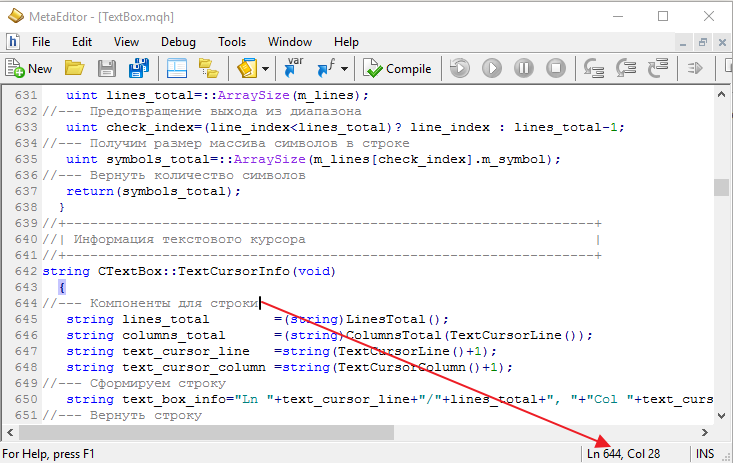Fig. 8. Position of the text cursor in the MetaEditor.

The listing below shows the code of the CTextBox::TextCursorInfo() method, which returns a string in the format as in the screenshot above. Also shown are the additional methods which can be used to get the number of lines and characters in the specified line, as well as the current positions of the text cursor.

```class CTextBox : public CElement
{
private:
//--- Returns the index of the (1) line, (2) character where the text cursor is located,
//    (3) the number of lines, (4) the number of characters in the specified line
uint              TextCursorLine(void)                      { return(m_text_cursor_y_pos);      }
uint              TextCursorColumn(void)                    { return(m_text_cursor_x_pos);      }
uint              LinesTotal(void)                          { return(::ArraySize(m_lines));     }
uint              ColumnsTotal(const uint line_index);
//--- Information about the text cursor (line/number of lines, column/number of columns)
string            TextCursorInfo(void);
};
//+------------------------------------------------------------------+
//| Returns the number of characters in the specified line           |
//+------------------------------------------------------------------+
uint CTextBox::ColumnsTotal(const uint line_index)
{
//--- Get the size of the lines array
uint lines_total=::ArraySize(m_lines);
//--- Prevention of exceeding the array size
uint check_index=(line_index<lines_total)? line_index : lines_total-1;
//--- Get the size of the array of characters in the line
uint symbols_total=::ArraySize(m_lines[check_index].m_symbol);
//--- Return the number of characters
return(symbols_total);
}
//+------------------------------------------------------------------+
//| Information about the text cursor                                |
//+------------------------------------------------------------------+
string CTextBox::TextCursorInfo(void)
{
//--- String components
string lines_total        =(string)LinesTotal();
string columns_total      =(string)ColumnsTotal(TextCursorLine());
string text_cursor_line   =string(TextCursorLine()+1);
string text_cursor_column =string(TextCursorColumn()+1);
//--- Generate the string
string text_box_info="Ln "+text_cursor_line+"/"+lines_total+", "+"Col "+text_cursor_column+"/"+columns_total;
//--- Return the string
return(text_box_info);
}
```

Now everything is ready to provide the description of the CTextBox::OnClickTextBox() method, which has been mentioned at the beginning of this section (see the code below). Here, at the very beginning, there is a check for the name of the object, where the left mouse button was clicked. If it turned out that the clicking was not on the text box, send a message that editing has ended (ON_END_EDIT event identifier) in case the text box is still active. After that, deactivate the text box and leave the method.

If the click was on this text box, then two more checks are following. The program leaves the method, if the Read-only mode is enabled or if the control is blocked. If one of the conditions is false, go to the main code of the method.

First of all, chart management using a keyboard is disabled. Then (1) get the current offset of the visible area of the control, (2) determine the relative coordinates of the point where the click occurred. The calculations in the main cycle of the method will also require the line height.

First, search for the line where the click occurred in a cycle. Searching for the character is started only when the calculated Y coordinate of the click is between the upper and lower boundaries of the line. If it turns out that this line contains no characters, the text cursor and horizontal scrollbar should be moved to the beginning of the line. This stops the cycle.

If the line contains characters, the second cycle starts, which searches for the character where the click has occurred. The search principle here is almost the same as in the case with lines. The only difference is that the character width is obtained in each iteration, since not all fonts have the same width for all characters. If the clicked character is found, set the text cursor to the character position and complete the search. If the character in this line was not found and the last character was reached, move the cursor to the last position of the line, where the character is not present yet, and complete the search.

Next, if the multiline mode is enabled, it is necessary to check if the text cursor (at least partially) exceeds the boundaries of the visible area of the text box along the Y axis. If it does, adjust the visibility area relative to the position of the text cursor. After that, flag the text box as activated and redraw it

And at the very end of the CTextBox::OnClickTextBox() method generate an event that indicates that the text box has been activated (ON_CLICK_TEXT_BOX event identifier). To provide an unambiguous identification, also send the (1) identifier of the control, (2) index of the control and — additionally — (3) information on the cursor position.

```class CTextBox : public CElement
{
private:
//--- Handling of the press on the element
bool              OnClickTextBox(const string clicked_object);
};
//+------------------------------------------------------------------+
//| Handling clicking the control                                    |
//+------------------------------------------------------------------+
bool CTextBox::OnClickTextBox(const string clicked_object)
{
//--- Leave, if it has a different object name
if(m_canvas.Name()!=clicked_object)
{
//--- Send a message about the end of the line editing mode in the text box, if the text box was active
if(m_text_edit_state)
::EventChartCustom(m_chart_id,ON_END_EDIT,CElementBase::Id(),CElementBase::Index(),TextCursorInfo());
//--- Deactivate the text box
DeactivateTextBox();
return(false);
}
//--- Leave, if (1) the Read-only mode is enabled or if (2) the control is blocked
return(true);
//--- Disable chart management
m_chart.SetInteger(CHART_KEYBOARD_CONTROL,false);
//--- Get the offset along the X and Y axes
int xoffset=(int)m_canvas.GetInteger(OBJPROP_XOFFSET);
int yoffset=(int)m_canvas.GetInteger(OBJPROP_YOFFSET);
//--- Determine the text edit box coordinates below the mouse cursor
int x =m_mouse.X()-m_canvas.X()+xoffset;
int y =m_mouse.Y()-m_canvas.Y()+yoffset;
//--- Get the line height
int line_height=(int)LineHeight();
//--- Get the size of the lines array
uint lines_total=::ArraySize(m_lines);
//--- Determine the clicked character
for(uint l=0; l<lines_total; l++)
{
//--- Set the initial coordinates for checking the condition
int x_offset=m_text_x_offset;
int y_offset=m_text_y_offset+((int)l*line_height);
//--- Checking the condition along the Y axis
bool y_pos_check=(l<lines_total-1)?(y>=y_offset && y<y_offset+line_height) : y>=y_offset;
//--- If the click was not on this line, go to the next
if(!y_pos_check)
continue;
//--- Get the size of the array of characters
uint symbols_total=::ArraySize(m_lines[l].m_width);
//--- If this is an empty line, move the cursor to the specified position and leave the cycle
if(symbols_total<1)
{
SetTextCursor(0,l);
HorizontalScrolling(0);
break;
}
//--- Find the character that was clicked
for(uint s=0; s<symbols_total; s++)
{
//--- If the character is found, move the cursor to the specified position and leave the cycle
if(x>=x_offset && x<x_offset+m_lines[l].m_width[s])
{
SetTextCursor(s,l);
l=lines_total;
break;
}
//--- Add the width of the current character for the next check
x_offset+=m_lines[l].m_width[s];
//--- If this is the last character, move the cursor to the end of the line and leave the cycle
if(s==symbols_total-1 && x>x_offset)
{
SetTextCursor(s+1,l);
l=lines_total;
break;
}
}
}
//--- If the multiline text box mode is enabled
if(m_multi_line_mode)
{
//--- Get the boundaries of the visible portion of the text box
CalculateYBoundaries();
//--- Get the Y coordinate of the cursor
CalculateTextCursorY();
//--- Move the scrollbar if the text cursor leaves the visibility area
if(m_text_cursor_y<=m_y_limit)
VerticalScrolling(CalculateScrollThumbY());
else
{
if(m_text_cursor_y+(int)LineHeight()>=m_y2_limit)
VerticalScrolling(CalculateScrollThumbY2());
}
}
//--- Activate the text box
m_text_edit_state=true;
//--- Update the text and the cursor
DrawTextAndCursor(true);
//--- Send a message about it
::EventChartCustom(m_chart_id,ON_CLICK_TEXT_BOX,CElementBase::Id(),CElementBase::Index(),TextCursorInfo());
return(true);
}
```

### Entering a character

Now consider the CTextBox::OnPressedKey() method. It handles the key presses, and if it turns out that the pressed key contains a character, then it must be added to the line at the current position of the text cursor. Additional methods will be required to increase the sizes of arrays in the KeySymbolOptions structure, adding the character entered in the text box to the arrays, as well as the width of the character to the added element of the arrays.

A rather simple CTextBox::ArraysResize() method will be used for resizing arrays in numerous methods of the CTextBox class:

```class CTextBox : public CElement
{
private:
//--- Resizes the arrays of properties for the specified line
void              ArraysResize(const uint line_index,const uint new_size);
};
//+------------------------------------------------------------------+
//| Resizes the arrays of properties for the specified line          |
//+------------------------------------------------------------------+
void CTextBox::ArraysResize(const uint line_index,const uint new_size)
{
//--- Get the size of the lines array
uint lines_total=::ArraySize(m_lines);
//--- Prevention of exceeding the array size
uint l=(line_index<lines_total)? line_index : lines_total-1;
//--- Set the size of the arrays of the structure
::ArrayResize(m_lines[line_index].m_width,new_size);
::ArrayResize(m_lines[line_index].m_symbol,new_size);
}
```

The CTextBox::AddSymbol() method is intended for adding a newly entered character to the text box. Let's look at it more carefully. When entering a new character, the sizes of arrays should be increased by one element. The current position of the text cursor can be at any character of the string. Therefore, before adding a character to the array, it is first necessary to shift all the characters to the right of the current text cursor location by one index to the right. After that, store the entered character at the cursor position of the text cursor. At the end of the method, shift the text cursor to the right by one character

```class CTextBox : public CElement
{
private:
//--- Adds a character and its properties to the arrays of the structure
};
//+------------------------------------------------------------------+
//| Adds character and its properties to the arrays of the structure |
//+------------------------------------------------------------------+
{
//--- Get the size of the array of characters
uint symbols_total=::ArraySize(m_lines[m_text_cursor_y_pos].m_symbol);
//--- Resize the arrays
ArraysResize(m_text_cursor_y_pos,symbols_total+1);
//--- Shift all characters from the end of the array to the index of the added character
for(uint i=symbols_total; i>m_text_cursor_x_pos; i--)
{
m_lines[m_text_cursor_y_pos].m_symbol[i] =m_lines[m_text_cursor_y_pos].m_symbol[i-1];
m_lines[m_text_cursor_y_pos].m_width[i]  =m_lines[m_text_cursor_y_pos].m_width[i-1];
}
//--- Get the width of the character
int width=m_canvas.TextWidth(key_symbol);
//--- Add the character to the vacated element
m_lines[m_text_cursor_y_pos].m_symbol[m_text_cursor_x_pos] =key_symbol;
m_lines[m_text_cursor_y_pos].m_width[m_text_cursor_x_pos]  =width;
//--- Increase the cursor position counter
m_text_cursor_x_pos++;
}
```

The listing below shows the code of the CTextBox::OnPressedKey() method. If the text box is activated, then try to get the character by the key code passed to the method. If the pressed key does not contain a character, then the program leaves the method. If there is a character, then it is added to the arrays along with its properties. The text box size might have changed when entering a character, so the new values are calculated and set. After that, get the boundaries of the text box and the current coordinate of the text cursor. If the cursor goes beyond the right edge of the text box, adjust the position of the horizontal scrollbar thumb. After that the text box is redrawn with force display (true) of the text cursor. At the very end of the CTextBox::OnPressedKey() method, an event of moving the text cursor (ON_MOVE_TEXT_CURSOR) is generated with the control identifier, control index and additional information on the location of the text cursor.

```class CTextBox : public CElement
{
private:
//--- Handling a keypress
bool              OnPressedKey(const long key_code);
};
//+------------------------------------------------------------------+
//| Handling a keypress                                              |
//+------------------------------------------------------------------+
bool CTextBox::OnPressedKey(const long key_code)
{
//--- Leave, if the text box is not activated
if(!m_text_edit_state)
return(false);
//--- Get the key character
string pressed_key=m_keys.KeySymbol(key_code);
//--- Leave, if there is no character
if(pressed_key=="")
return(false);
//--- Add the character and its properties
//--- Calculate the size of the text box
CalculateTextBoxSize();
//--- Set the new size to the text box
ChangeTextBoxSize(true,true);
//--- Get the boundaries of the visible portion of the text box
CalculateXBoundaries();
//--- Get the X coordinate of the cursor
CalculateTextCursorX();
//--- Move the scrollbar if the text cursor leaves the visibility area
if(m_text_cursor_x>=m_x2_limit)
HorizontalScrolling(CalculateScrollThumbX2());
//--- Update the text in the text box
DrawTextAndCursor(true);
//--- Send a message about it
::EventChartCustom(m_chart_id,ON_MOVE_TEXT_CURSOR,CElementBase::Id(),CElementBase::Index(),TextCursorInfo());
return(true);
}
```

### Handling the pressing of the Backspace key

Now consider the situation when a character is deleted by pressing the Backspace key. In this case, the event handler of the Multiline Text box control will call the CTextBox::OnPressedKeyBackspace() method. Its operation will require additional methods, which had not been considered before. First, their code will be presented.

Characters are deleted using the CTextBox::DeleteSymbol() method. At the beginning, it checks if the current line contains at least one character. If not anymore, then the text cursor is placed at the beginning of the line and the method is exited. If there still are some characters, then get the position of the previous character. This will be the index, starting from which all characters are to be shifted right by one element. After that the text cursor is also shifted to the left by one position. And at the end of the method, the sizes of arrays are decreased by one element.

```class CTextBox : public CElement
{
private:
//--- Deletes a character
void              DeleteSymbol(void);
};
//+------------------------------------------------------------------+
//| Deletes a character                                              |
//+------------------------------------------------------------------+
void CTextBox::DeleteSymbol(void)
{
//--- Get the size of the array of characters
uint symbols_total=::ArraySize(m_lines[m_text_cursor_y_pos].m_symbol);
//--- If the array is empty
if(symbols_total<1)
{
//--- Set the cursor to the zero position of the cursor line
SetTextCursor(0,m_text_cursor_y_pos);
return;
}
//--- Get the position of the previous character
int check_pos=(int)m_text_cursor_x_pos-1;
//--- Leave, if out of range
if(check_pos<0)
return;
//--- Shift all characters by one element to the right from the index of the deleted character to the end of the array
for(uint i=check_pos; i<symbols_total-1; i++)
{
m_lines[m_text_cursor_y_pos].m_symbol[i] =m_lines[m_text_cursor_y_pos].m_symbol[i+1];
m_lines[m_text_cursor_y_pos].m_width[i]  =m_lines[m_text_cursor_y_pos].m_width[i+1];
}
//--- Decrease the cursor position counter
m_text_cursor_x_pos--;
//--- Resize the arrays
ArraysResize(m_text_cursor_y_pos,symbols_total-1);
}
```

If the text cursor is at the beginning of the line, and it is not the first line, it is necessary to delete the current line and move all lower lines up by one position. If the deleted line has characters, they need to be appended to the line, which is one position higher. Another additional method will be used for this operation — CTextBox::ShiftOnePositionUp(). An auxiliary CTextBox::LineCopy() method will also be required to slightly facilitate the copying of lines.

```class CTextBox : public CElement
{
private:
//--- Makes a copy of the specified (source) line to a new location (destination)
void              LineCopy(const uint destination,const uint source);
};
//+------------------------------------------------------------------+
//| Resizes the arrays of properties for the specified line          |
//+------------------------------------------------------------------+
void CTextBox::LineCopy(const uint destination,const uint source)
{
::ArrayCopy(m_lines[destination].m_width,m_lines[source].m_width);
::ArrayCopy(m_lines[destination].m_symbol,m_lines[source].m_symbol);
}
```

The code of the CTextBox::ShiftOnePositionUp() method is presented below. The first cycle of the method shifts all lines below the current position of the cursor up by one position. In the first iteration, it is necessary to check if the line contains characters, and if it does, store them to append to the previous line Once the lines have been shifted, the array of lines is decreased by one element. The text cursor is moved to the end of the previous line.

The last block of the CTextBox::ShiftOnePositionUp() method is intended to append characters of the deleted line to the previous line. If there is a line to be appended, then use the ::StringToCharArray() function to transfer it to a temporary array of uchar type in the form of character codes. Then, increase the array of the current line by the number of added characters. And as a finishing operation, alternately add the characters and their properties to the arrays. Conversion of the character codes from the temporary array of uchar type is performed using the ::CharToString() function

```class CTextBox : public CElement
{
private:
//--- Shifts the lines up by one position
void              ShiftOnePositionUp(void);
};
//+------------------------------------------------------------------+
//| Shifts the lines up by one position                              |
//+------------------------------------------------------------------+
void CTextBox::ShiftOnePositionUp(void)
{
//--- Get the size of the lines array
uint lines_total=::ArraySize(m_lines);
//--- Shift the lines up starting from the next element by one position
for(uint i=m_text_cursor_y_pos; i<lines_total-1; i++)
{
//--- In the first iteration
if(i==m_text_cursor_y_pos)
{
//--- Get the size of the array of characters
uint symbols_total=::ArraySize(m_lines[i].m_symbol);
//--- If there are characters in this line, store them in order to append to the previous line
m_temp_input_string=(symbols_total>0)? CollectString(i) : "";
}
//--- Index of the next element of the lines array
uint next_index=i+1;
//--- Get the size of the array of characters
uint symbols_total=::ArraySize(m_lines[next_index].m_symbol);
//--- Resize the arrays
ArraysResize(i,symbols_total);
//--- make a copy of the line
LineCopy(i,next_index);
}
//--- Resize the lines array
uint new_size=lines_total-1;
::ArrayResize(m_lines,new_size);
//--- Decrease the lines counter
m_text_cursor_y_pos--;
//--- Get the size of the array of characters
uint symbols_total=::ArraySize(m_lines[m_text_cursor_y_pos].m_symbol);
//--- Move the cursor to the end
m_text_cursor_x_pos=symbols_total;
//--- Get the X coordinate of the cursor
CalculateTextCursorX();
//--- If there is a line that must be appended to the previous one
if(m_temp_input_string!="")
{
//--- Transfer the line to array
uchar array[];
int total=::StringToCharArray(m_temp_input_string,array)-1;
//--- Get the size of the array of characters
symbols_total=::ArraySize(m_lines[m_text_cursor_y_pos].m_symbol);
//--- Resize the arrays
new_size=symbols_total+total;
ArraysResize(m_text_cursor_y_pos,new_size);
//--- Add the data to the arrays of the structure
for(uint i=m_text_cursor_x_pos; i<new_size; i++)
{
m_lines[m_text_cursor_y_pos].m_symbol[i] =::CharToString(array[i-m_text_cursor_x_pos]);
m_lines[m_text_cursor_y_pos].m_width[i]  =m_canvas.TextWidth(m_lines[m_text_cursor_y_pos].m_symbol[i]);
}
}
}
```

Once all the auxiliary methods are ready, the code of the main CTextBox::OnPressedKeyBackspace() method does not seem too complicated. Here, at the very beginning, check if the Backspace key was pressed and if the text box is activated. If the checks are passed, then see the position the text cursor is currently located at. If it is not at the beginning of a line at the moment, delete the previous character. If, however, it is at the beginning of the line and it is not the first line, then shift all lower lines up by one position, deleting the current line

After that, the new sizes for the text box are calculated and set. The boundaries and coordinates of the text cursor are obtained. Adjust the scrollbar thumb, if the text cursor leaves the visible area. And, finally, the control is redrawn with forced display of the text cursor and a message about shifting the cursor is generated.

```class CTextBox : public CElement
{
private:
//--- Handling the pressing of the Backspace key
bool              OnPressedKeyBackspace(const long key_code);
};
//+------------------------------------------------------------------+
//| Handling the pressing of the Backspace key                       |
//+------------------------------------------------------------------+
bool CTextBox::OnPressedKeyBackspace(const long key_code)
{
//--- Leave, if it is not the Backspace key of if the text box is not activated
if(key_code!=KEY_BACKSPACE || !m_text_edit_state)
return(false);
//--- Delete the character, if the position is greater than zero
if(m_text_cursor_x_pos>0)
DeleteSymbol();
//--- Delete the line, if the position is zero and it is not the first line
else if(m_text_cursor_y_pos>0)
{
//--- Shift the lines up by one position
ShiftOnePositionUp();
}
//--- Calculate the size of the text box
CalculateTextBoxSize();
//--- Set the new size to the text box
ChangeTextBoxSize(true,true);
//--- Get the boundaries of the visible portion of the text box
CalculateBoundaries();
//--- Get the X and Y coordinates of the cursor
CalculateTextCursorX();
CalculateTextCursorY();
//--- Move the scrollbar if the text cursor leaves the visibility area
if(m_text_cursor_x<=m_x_limit)
HorizontalScrolling(CalculateScrollThumbX());
else
{
if(m_text_cursor_x>=m_x2_limit)
HorizontalScrolling(CalculateScrollThumbX2());
}
//--- Move the scrollbar if the text cursor leaves the visibility area
if(m_text_cursor_y<=m_y_limit)
VerticalScrolling(CalculateScrollThumbY());
else
VerticalScrolling(m_scrollv.CurrentPos());
//--- Update the text in the text box
DrawTextAndCursor(true);
//--- Send a message about it
::EventChartCustom(m_chart_id,ON_MOVE_TEXT_CURSOR,CElementBase::Id(),CElementBase::Index(),TextCursorInfo());
return(true);
}
```

### Handling the pressing of the Enter key

If the multiline mode is enabled and the Enter key is pressed, it is necessary to add a new line, and all lines below the current position of the text cursor must be shifted down by one position. Shifting the lines here will require a separate auxiliary CTextBox::ShiftOnePositionDown() method, as well as an additional method for clearing the lines - CTextBox::ClearLine().

```class CTextBox : public CElement
{
private:
//--- Clears the specified line
void              ClearLine(const uint line_index);
};
//+------------------------------------------------------------------+
//| Clears the specified line                                        |
//+------------------------------------------------------------------+
void CTextBox::ClearLine(const uint line_index)
{
::ArrayFree(m_lines[line_index].m_width);
::ArrayFree(m_lines[line_index].m_symbol);
}
```

Now, let's examine the algorithm of CTextBox::ShiftOnePositionDown() method in details. First of all, it is necessary to store the number of characters in the line, where the Enter key had been pressed. This, as well as the position in the line, where the text cursor was located, define the how the algorithm of the CTextBox::ShiftOnePositionDown() method will be processed. After that, move the text cursor to a new line and increase the size of the lines array by one element. Then all lines starting from the current line must be shifted down by one position in a cycle starting from the end of the array. In the last iteration, if the line where the Enter key had been pressed contains no characters, then it is necessary to clear the line where the text cursor is currently located. The cleared line is a copy of the line, the content of which is already present on the next line as a result of shifting down by one position.

At the beginning of the method, we stored the number of characters in the line where the Enter key had been pressed. If it turns out that the line contained characters, it is necessary to find out where the text cursor was located at that moment. And if it turns out that it was not at the end of the line, then it is necessary to calculate the number of characters to be moved to the new line, starting from the current position of the text cursor to the end of the line. For these purposes, a temporary array is used here, where the characters will be copied, which will later be moved to the new line.

```class CTextBox : public CElement
{
private:
//--- Shifts the lines down by one position
void              ShiftOnePositionDown(void);
};
//+------------------------------------------------------------------+
//| Shifts the lines down by one position                            |
//+------------------------------------------------------------------+
void CTextBox::ShiftOnePositionDown(void)
{
//--- Get the size of the array of characters from the line, where the Enter key was pressed
uint pressed_line_symbols_total=::ArraySize(m_lines[m_text_cursor_y_pos].m_symbol);
//--- Increase the lines counter
m_text_cursor_y_pos++;
//--- Get the size of the lines array
uint lines_total=::ArraySize(m_lines);
//--- Increase the array by one element
uint new_size=lines_total+1;
::ArrayResize(m_lines,new_size);
//--- Shift the lines down starting from the current position by one item (from the end of the array)
for(uint i=lines_total; i>m_text_cursor_y_pos; i--)
{
//--- Index of the previous element of the lines array
uint prev_index=i-1;
//--- Get the size of the array of characters
uint symbols_total=::ArraySize(m_lines[prev_index].m_symbol);
//--- Resize the arrays
ArraysResize(i,symbols_total);
//--- make a copy of the line
LineCopy(i,prev_index);
//--- Clear the new line
if(prev_index==m_text_cursor_y_pos && pressed_line_symbols_total<1)
ClearLine(prev_index);
}
//--- If the Enter key wan not pressed on an empty line
if(pressed_line_symbols_total>0)
{
//--- Index of the line, where the Enter key was pressed
uint prev_line_index=m_text_cursor_y_pos-1;
//--- Array for the copies of the characters starting from the current position of the cursor to the end of the line
string array[];
//--- Set the size of the array equal to the number of characters that must be moved to the new line
uint new_line_size=pressed_line_symbols_total-m_text_cursor_x_pos;
::ArrayResize(array,new_line_size);
//--- Copy the characters to be moved to the new line into an array
for(uint i=0; i<new_line_size; i++)
array[i]=m_lines[prev_line_index].m_symbol[m_text_cursor_x_pos+i];
//--- Resize the arrays of the structure for the line where the Enter key was pressed
ArraysResize(prev_line_index,pressed_line_symbols_total-new_line_size);
//--- Resize the arrays of the structure for the new line
ArraysResize(m_text_cursor_y_pos,new_line_size);
//--- Add the data to the arrays of the structure for the new line
for(uint k=0; k<new_line_size; k++)
{
m_lines[m_text_cursor_y_pos].m_symbol[k] =array[k];
m_lines[m_text_cursor_y_pos].m_width[k]  =m_canvas.TextWidth(array[k]);
}
}
}
```

Everything is now ready to handle the pressing of the Enter key. Now consider the CTextBox::OnPressedKeyEnter() method. At the very beginning, check if the Enter key is pressed and in the text box is activated. Then, if all checks are passed, and if it is a single-line text box, simply finish working with it. To do this, deactivate by sending an event with the ON_END_EDIT identifier and leave the method.

If the multiline mode is enabled, then all lower lines are shifted down by one position starting from the current position of the text cursor. After that the text box sizes are adjusted and a check is made if the text cursor exceeds the lower boundary of the visibility area. In addition, the text cursor is placed at the beginning of the line. At the end of the method, the text box is redrawn and a message is sent, saying that the text cursor was moved.

```class CTextBox : public CElement
{
private:
//--- Handling the pressing of the Enter key
bool              OnPressedKeyEnter(const long key_code);
};
//+------------------------------------------------------------------+
//| Handling the pressing of the Enter key                           |
//+------------------------------------------------------------------+
bool CTextBox::OnPressedKeyEnter(const long key_code)
{
//--- Leave, if it is not the Enter key of if the text box is not activated
if(key_code!=KEY_ENTER || !m_text_edit_state)
return(false);
//--- If the multiline mode is disabled
if(!m_multi_line_mode)
{
//--- Deactivate the text box
DeactivateTextBox();
//--- Send a message about it
::EventChartCustom(m_chart_id,ON_END_EDIT,CElementBase::Id(),CElementBase::Index(),TextCursorInfo());
return(false);
}
//--- Shift the lines down by one position
ShiftOnePositionDown();
//--- Calculate the size of the text box
CalculateTextBoxSize();
//--- Set the new size to the text box
ChangeTextBoxSize();
//--- Get the boundaries of the visible portion of the text box
CalculateYBoundaries();
//--- Get the Y coordinate of the cursor
CalculateTextCursorY();
//--- Move the scrollbar if the text cursor leaves the visibility area
if(m_text_cursor_y+(int)LineHeight()>=m_y2_limit)
VerticalScrolling(CalculateScrollThumbY2());
//--- Move the cursor to the beginning of the line
SetTextCursor(0,m_text_cursor_y_pos);
//--- Move the scrollbar to the beginning
HorizontalScrolling(0);
//--- Update the text in the text box
DrawTextAndCursor(true);
//--- Send a message about it
::EventChartCustom(m_chart_id,ON_MOVE_TEXT_CURSOR,CElementBase::Id(),CElementBase::Index(),TextCursorInfo());
return(true);
}
```

### Handling the pressing of the Left and Right keys

By pressing the Left or Right key, the text cursor is moved by one character in the corresponding direction. To accomplish this, an additional CTextBox::CorrectingTextCursorXPos() method will be required first, which will adjust the location of the text cursor. This method will also be used in other methods of the class.

```class CTextBox : public CElement
{
private:
//--- Adjusting the text cursor along the X axis
void              CorrectingTextCursorXPos(const int x_pos=WRONG_VALUE);
};
//+------------------------------------------------------------------+
//| Adjusting the text cursor along the X axis                       |
//+------------------------------------------------------------------+
void CTextBox::CorrectingTextCursorXPos(const int x_pos=WRONG_VALUE)
{
//--- Get the size of the array of characters
uint symbols_total=::ArraySize(m_lines[m_text_cursor_y_pos].m_width);
//--- Determine the cursor position
uint text_cursor_x_pos=0;
//--- If the position is available
if(x_pos!=WRONG_VALUE)
text_cursor_x_pos=(x_pos>(int)symbols_total-1)? symbols_total : x_pos;
//--- If the position is not available, set the cursor to the end of the line
else
text_cursor_x_pos=symbols_total;
//--- Zero position, if the line contains no characters
m_text_cursor_x_pos=(symbols_total<1)? 0 : text_cursor_x_pos;
//--- Get the X coordinate of the cursor
CalculateTextCursorX();
}
```

The code below shows the code of the CTextBox::OnPressedKeyLeft() method for handling the pressing of the Left key. The program leaves the method if another key was pressed or if the text box is not activated, and also if the Ctrl is pressed at the moment. Handling the simultaneous pressing keys with the Ctrl key will be considered in another section of the article.

If the first checks are passed, then see the position of the text cursor. If it is located not at the beginning of the line, then shift it to the previous character. If it is at the beginning of the line, and if this line is not the first, then the text cursor must be moved to the end of the previous line. After that, adjust the thumbs of the horizontal and vertical scrollbars, redraw the text box and send a message about moving the text cursor.

```class CTextBox : public CElement
{
private:
//--- Handling the pressing of the Left key
bool              OnPressedKeyLeft(const long key_code);
};
//+------------------------------------------------------------------+
//| Handling the pressing of the Left key                            |
//+------------------------------------------------------------------+
bool CTextBox::OnPressedKeyLeft(const long key_code)
{
//--- Leave, if it is not the Left key or if the Ctrl key is pressed or if text box is not activated
if(key_code!=KEY_LEFT || m_keys.KeyCtrlState() || !m_text_edit_state)
return(false);
//--- If the text cursor position is greater than zero
if(m_text_cursor_x_pos>0)
{
//--- Shift it to the previous character
m_text_cursor_x-=m_lines[m_text_cursor_y_pos].m_width[m_text_cursor_x_pos-1];
//--- Decrease the characters counter
m_text_cursor_x_pos--;
}
else
{
//--- If this is not the first line
if(m_text_cursor_y_pos>0)
{
//--- Move to the end of the previous line
m_text_cursor_y_pos--;
CorrectingTextCursorXPos();
}
}
//--- Get the boundaries of the visible portion of the text box
CalculateBoundaries();
//--- Get the Y coordinate of the cursor
CalculateTextCursorY();
//--- Move the scrollbar if the text cursor leaves the visibility area
if(m_text_cursor_x<=m_x_limit)
HorizontalScrolling(CalculateScrollThumbX());
else
{
//--- Get the size of the array of characters
uint symbols_total=::ArraySize(m_lines[m_text_cursor_y_pos].m_symbol);
//---
if(m_text_cursor_x_pos==symbols_total && m_text_cursor_x>=m_x2_limit)
HorizontalScrolling(CalculateScrollThumbX2());
}
//--- Move the scrollbar if the text cursor leaves the visibility area
if(m_text_cursor_y<=m_y_limit)
VerticalScrolling(CalculateScrollThumbY());
//--- Update the text in the text box
DrawTextAndCursor(true);
//--- Send a message about it
::EventChartCustom(m_chart_id,ON_MOVE_TEXT_CURSOR,CElementBase::Id(),CElementBase::Index(),TextCursorInfo());
return(true);
}
```

Now consider the code of the CTextBox::OnPressedKeyRight() method for handling the pressing of the Right key. Here, the checks for the code of the pressed key, the text box state and the Ctrl key must also be passed at the beginning of the method. Then see, if the text cursor is at the end of the line. If not, then move the text cursor to the right by one character. If the text cursor is located at the end of the line, then see if this line is the last. If not, then move the text cursor to the beginning of the next line.

Then (1) adjust the scrollbar thumbs in case the text cursor goes beyond the text box visibility area, (2) redraw the control and (3) send a message about moving the text cursor.

```class CTextBox : public CElement
{
private:
//--- Handling the pressing of the Right key
bool              OnPressedKeyRight(const long key_code);
};
//+------------------------------------------------------------------+
//| Handling the pressing of the Right key                           |
//+------------------------------------------------------------------+
bool CTextBox::OnPressedKeyRight(const long key_code)
{
//--- Leave, if it is not the Right key or if the Ctrl key is pressed or if text box is not activated
if(key_code!=KEY_RIGHT || m_keys.KeyCtrlState() || !m_text_edit_state)
return(false);
//--- Get the size of the array of characters
uint symbols_total=::ArraySize(m_lines[m_text_cursor_y_pos].m_width);
//--- If this is the end of the line
if(m_text_cursor_x_pos<symbols_total)
{
//--- Shift the position of the text cursor to the next character
m_text_cursor_x+=m_lines[m_text_cursor_y_pos].m_width[m_text_cursor_x_pos];
//--- Increase the character counter
m_text_cursor_x_pos++;
}
else
{
//--- Get the size of the lines array
uint lines_total=::ArraySize(m_lines);
//--- If this is not the last line
if(m_text_cursor_y_pos<lines_total-1)
{
//--- Move the cursor to the beginning of the next line
m_text_cursor_x=m_text_x_offset;
SetTextCursor(0,++m_text_cursor_y_pos);
}
}
//--- Get the boundaries of the visible portion of the text box
CalculateBoundaries();
//--- Get the Y coordinate of the cursor
CalculateTextCursorY();
//--- Move the scrollbar if the text cursor leaves the visibility area
if(m_text_cursor_x>=m_x2_limit)
HorizontalScrolling(CalculateScrollThumbX2());
else
{
if(m_text_cursor_x_pos==0)
HorizontalScrolling(0);
}
//--- Move the scrollbar if the text cursor leaves the visibility area
if(m_text_cursor_y+(int)LineHeight()>=m_y2_limit)
VerticalScrolling(CalculateScrollThumbY2());
//--- Update the text in the text box
DrawTextAndCursor(true);
//--- Send a message about it
::EventChartCustom(m_chart_id,ON_MOVE_TEXT_CURSOR,CElementBase::Id(),CElementBase::Index(),TextCursorInfo());
return(true);
}
```

### Handling the pressing of the Up and Down keys

Pressing the Up and Down keys causes the text cursor to move up and down the lines. The CTextBox::OnPressedKeyUp() and CTextBox::OnPressedKeyDown() methods are designed to handle the pressing of those keys. The code of only one of them will be provided here, as the only difference between them lies in merely two lines of code.

At the beginning of the code, it is necessary to pass three checks. The program leaves the method if (1) it is a single-line text box or if (2) another key is pressed or of (3) the text box is not activated. If the current position of the text cursor is not on the first line, then move it to the previous line (to the next line in the CTextBox::OnPressedKeyDown() method) with adjustment for the number of characters in the case of going beyond the lines array range.

After that, check if the text cursor is outside the visible area of the text box and adjust the scrollbar thumbs, if necessary. Here, the only difference between the two methods is that the CTextBox::OnPressedKeyUp() method checks exceeding the upper border, and the CTextBox::OnPressedKeyDown() method — exceeding the lower border. At the very end, the text box is redrawn and the message about moving the text cursor is sent.

```class CTextBox : public CElement
{
private:
//--- Handling the pressing of the Up key
bool              OnPressedKeyUp(const long key_code);
//--- Handling the pressing of the Down key
bool              OnPressedKeyDown(const long key_code);
};
//+------------------------------------------------------------------+
//| Handling the pressing of the Up key                              |
//+------------------------------------------------------------------+
bool CTextBox::OnPressedKeyUp(const long key_code)
{
//--- Leave, if the multiline mode is disabled
if(!m_multi_line_mode)
return(false);
//--- Leave, if it is not the Up key of if the text box is not activated
if(key_code!=KEY_UP || !m_text_edit_state)
return(false);
//--- Get the size of the lines array
uint lines_total=::ArraySize(m_lines);
//--- If not exceeding the array range
if(m_text_cursor_y_pos-1<lines_total)
{
//--- Move to the previous line
m_text_cursor_y_pos--;
//--- Adjusting the text cursor along the X axis
CorrectingTextCursorXPos(m_text_cursor_x_pos);
}
//--- Get the boundaries of the visible portion of the text box
CalculateBoundaries();
//--- Get the Y coordinate of the cursor
CalculateTextCursorY();
//--- Move the scrollbar if the text cursor leaves the visibility area
if(m_text_cursor_x<=m_x_limit)
HorizontalScrolling(CalculateScrollThumbX());
//--- Move the scrollbar if the text cursor leaves the visibility area
if(m_text_cursor_y<=m_y_limit)
VerticalScrolling(CalculateScrollThumbY());
//--- Update the text in the text box
DrawTextAndCursor(true);
//--- Send a message about it
::EventChartCustom(m_chart_id,ON_MOVE_TEXT_CURSOR,CElementBase::Id(),CElementBase::Index(),TextCursorInfo());
return(true);
}
```

### Handling the pressing of the Home and End keys

Pressing the Home and End keys moves the text cursor to the beginning and end of the line, respectively. The CTextBox::OnPressedKeyHome() and CTextBox::OnPressedKeyEnd() methods are designed to handle these events. Their code is provided in the listing below and it does not require any further explanation, as it is quite simple and features detailed comments.

```class CTextBox : public CElement
{
private:
//--- Handling the pressing of the Home key
bool              OnPressedKeyHome(const long key_code);
//--- Handling the pressing of the End key
bool              OnPressedKeyEnd(const long key_code);
};
//+------------------------------------------------------------------+
//| Handling the pressing of the Home key                            |
//+------------------------------------------------------------------+
bool CTextBox::OnPressedKeyHome(const long key_code)
{
//--- Leave, if it is not the Home key or if the Ctrl key is pressed or if the text box is not activated
if(key_code!=KEY_HOME || m_keys.KeyCtrlState() || !m_text_edit_state)
return(false);
//--- Move the cursor to the beginning of the current line
SetTextCursor(0,m_text_cursor_y_pos);
//--- Move the scrollbar to the first position
HorizontalScrolling(0);
//--- Update the text in the text box
DrawTextAndCursor(true);
//--- Send a message about it
::EventChartCustom(m_chart_id,ON_MOVE_TEXT_CURSOR,CElementBase::Id(),CElementBase::Index(),TextCursorInfo());
return(true);
}
//+------------------------------------------------------------------+
//| Handling the pressing of the End key                             |
//+------------------------------------------------------------------+
bool CTextBox::OnPressedKeyEnd(const long key_code)
{
//--- Leave, if it is not the End key or if the Ctrl key is pressed or if the text box is not activated
if(key_code!=KEY_END || m_keys.KeyCtrlState() || !m_text_edit_state)
return(false);
//--- Get the number of characters in the current line
uint symbols_total=::ArraySize(m_lines[m_text_cursor_y_pos].m_symbol);
//--- Move the cursor to the end of the current line
SetTextCursor(symbols_total,m_text_cursor_y_pos);
//--- Get the X coordinate of the cursor
CalculateTextCursorX();
//--- Get the boundaries of the visible portion of the text box
CalculateXBoundaries();
//--- Move the scrollbar if the text cursor leaves the visibility area
if(m_text_cursor_x>=m_x2_limit)
HorizontalScrolling(CalculateScrollThumbX2());
//--- Update the text in the text box
DrawTextAndCursor(true);
//--- Send a message about it
::EventChartCustom(m_chart_id,ON_MOVE_TEXT_CURSOR,CElementBase::Id(),CElementBase::Index(),TextCursorInfo());
return(true);
}
```

### Handling the simultaneous pressing of keys in combination with the Ctrl key

Now let us consider the methods for handling the following key combinations:

• 'Ctrl' + 'Left' – move the text cursor from the word to the word on the left.
• 'Ctrl' + 'Right' – move the text cursor from the word to the word on the right.
• 'Ctrl' + 'Home' – move the text cursor to the beginning of the first line.
• 'Ctrl' + 'End' – move the text cursor to the end of the last line.

As an example, only one of the methods will be considered — CTextBox::OnPressedKeyCtrlAndLeft(), for moving the text cursor from a word to a word on the left. At the beginning of the method there is a check for simultaneous pressing of the Ctrl and Left keys. If any of these keys is not pressed, the program leaves the method. In addition, the text box must be activated.

In case the current position of the text cursor is at the beginning of the line, and it is not the first line, move it to the end of the previous line. If the text cursor is not at the beginning of the current line, then it is necessary to find the beginning of an uninterrupted sequence of characters. The space character (' ') serves as the interrupt character. Here, in a cycle, move along the current line from right to left, and once the combination is found, when the next character is space and the current is any other character, then, if it is not the starting point, set the text cursor to that position.

After that, as in all other methods, there is a check if the text cursor is outside the visible area of the text box and the scrollbar thumbs are adjusted, if necessary. At the very end, the text box is redrawn and a message is sent, saying that the text cursor was moved.

```class CTextBox : public CElement
{
private:
//--- Handling the pressing of the Ctrl + Left keys
bool              OnPressedKeyCtrlAndLeft(const long key_code);
//--- Handling the pressing of the Ctrl + Right keys
bool              OnPressedKeyCtrlAndRight(const long key_code);
//--- Handling the simultaneous pressing of the Ctrl + Home keys
bool              OnPressedKeyCtrlAndHome(const long key_code);
//--- Handling the simultaneous pressing of the Ctrl + End keys
bool              OnPressedKeyCtrlAndEnd(const long key_code);
};
//+------------------------------------------------------------------+
//| Handling the simultaneous pressing of the Ctrl + Left keys       |
//+------------------------------------------------------------------+
bool CTextBox::OnPressedKeyCtrlAndLeft(const long key_code)
{
//--- Leave, if (1) it is not the Left key or if (2) the Ctrl key is not pressed or if (3) the text box is not activated
if(!(key_code==KEY_LEFT && m_keys.KeyCtrlState()) || !m_text_edit_state)
return(false);
//--- Space character
string SPACE=" ";
//--- Get the size of the lines array
uint lines_total=::ArraySize(m_lines);
//--- Get the number of characters in the current line
uint symbols_total=::ArraySize(m_lines[m_text_cursor_y_pos].m_symbol);
//--- If the cursor is at the beginning of the current line, and this is not the first line,
//    move the cursor to the end of the previous line
if(m_text_cursor_x_pos==0 && m_text_cursor_y_pos>0)
{
//--- Get the index of the previous line
uint prev_line_index=m_text_cursor_y_pos-1;
//--- Get the number of characters in the previous line
symbols_total=::ArraySize(m_lines[prev_line_index].m_symbol);
//--- Move the cursor to the end of the previous line
SetTextCursor(symbols_total,prev_line_index);
}
// --- If the cursor is at the beginning of the current line or the cursor is on the first line
else
{
//--- Find the beginning of a continuous sequence of characters (from right to left)
for(uint i=m_text_cursor_x_pos; i<=symbols_total; i--)
{
//--- Go to the next, if the cursor is at the end of the line
if(i==symbols_total)
continue;
//--- If this is the first character of the line
if(i==0)
{
//--- Set the cursor to the beginning of the line
SetTextCursor(0,m_text_cursor_y_pos);
break;
}
//--- If this is not the first character of the line
else
{
//--- If found the beginning of a continuous sequence for the first time.
//    The beginning is considered to be the space at the next index.
if(i!=m_text_cursor_x_pos &&
m_lines[m_text_cursor_y_pos].m_symbol[i]!=SPACE &&
m_lines[m_text_cursor_y_pos].m_symbol[i-1]==SPACE)
{
//--- Set the cursor to the beginning of a new continuous sequence
SetTextCursor(i,m_text_cursor_y_pos);
break;
}
}
}
}
//--- Get the boundaries of the visible portion of the text box
CalculateBoundaries();
//--- Get the X coordinate of the cursor
CalculateTextCursorX();
//--- Get the Y coordinate of the cursor
CalculateTextCursorY();
//--- Move the scrollbar if the text cursor leaves the visibility area
if(m_text_cursor_x<=m_x_limit)
HorizontalScrolling(CalculateScrollThumbX());
else
{
//--- Get the size of the array of characters
symbols_total=::ArraySize(m_lines[m_text_cursor_y_pos].m_symbol);
//---
if(m_text_cursor_x_pos==symbols_total && m_text_cursor_x>=m_x2_limit)
HorizontalScrolling(CalculateScrollThumbX2());
}
//--- Move the scrollbar if the text cursor leaves the visibility area
if(m_text_cursor_y<=m_y_limit)
VerticalScrolling(CalculateScrollThumbY());
//--- Update the text in the text box
DrawTextAndCursor(true);
//--- Send a message about it
::EventChartCustom(m_chart_id,ON_MOVE_TEXT_CURSOR,CElementBase::Id(),CElementBase::Index(),TextCursorInfo());
return(true);
}
```

The study of all other methods from the list at the beginning of this section is left to the reader.

### Integration of the control in the library engine

For the Multiline Text box control to work correctly, a private array will be required in the WindowElements structure of the CWndContainer class. Include the file with the CTextBox class in the WndContainer.mqh file:

```//+------------------------------------------------------------------+
//|                                                 WndContainer.mqh |
//|                        Copyright 2015, MetaQuotes Software Corp. |
//|                                              https://www.mql5.com |
//+------------------------------------------------------------------+
#include "Controls\TextBox.mqh"
```

Add a private array for the new control to the WindowElements structure:

```//+------------------------------------------------------------------+
//| Class for storing all interface objects                          |
//+------------------------------------------------------------------+
class CWndContainer
{
protected:
//--- Structure of the element arrays
struct WindowElements
{
//--- Multiline text boxes
CTextBox         *m_text_boxes[];
};
//--- Array of element arrays for each window
WindowElements    m_wnd[];
};
```

Since the CTextBox type controls are composite and contain controls of other types (in this case, scrollbars), a method is required, where pointers to these controls will be distributed to the corresponding private arrays. The listing below shows the code of the CWndContainer::AddTextBoxElements() method, which is designed for this purpose. This method is called in the same place as any other similar method, that is, in CWndContainer::AddToElementsArray().

```class CWndContainer
{
private:
//--- Stores pointers to objects of the multiline text box
};
//+------------------------------------------------------------------+
//| Stores pointers to objects of the multiline text box             |
//+------------------------------------------------------------------+
{
//--- Leave, if this is not a multiline text box
if(dynamic_cast<CTextBox *>(&object)==NULL)
return(false);
//--- Get the pointer to the control
CTextBox *tb=::GetPointer(object);
for(int i=0; i<2; i++)
{
int size=::ArraySize(m_wnd[window_index].m_elements);
::ArrayResize(m_wnd[window_index].m_elements,size+1);
if(i==0)
{
//--- Get the scrollbar pointer
CScrollV *sv=tb.GetScrollVPointer();
m_wnd[window_index].m_elements[size]=sv;
//--- Add the pointer to the private array
}
else if(i==1)
{
CScrollH *sh=tb.GetScrollHPointer();
m_wnd[window_index].m_elements[size]=sh;
//--- Add the pointer to the private array
}
}
//--- Add the pointer to the private array
return(true);
}
```

Now it is necessary to make certain addition to the CWndEvents::OnTimerEvent() method. Remember that the graphical interface is redrawn only when the mouse cursor moves and it is suspended a certain time after the mouse cursor movement stops. An exception should be made for controls of the CTextBox type. Otherwise, the text cursor will not blink when the text box is activated.

```//+------------------------------------------------------------------+
//| Timer                                                            |
//+------------------------------------------------------------------+
void CWndEvents::OnTimerEvent(void)
{
//--- Leave, if mouse cursor is at rest (difference between call is >300 ms) and the left mouse button is released
if(m_mouse.GapBetweenCalls()>300 && !m_mouse.LeftButtonState())
{
int text_boxes_total=CWndContainer::TextBoxesTotal(m_active_window_index);
for(int e=0; e<text_boxes_total; e++)
m_wnd[m_active_window_index].m_text_boxes[e].OnEventTimer();
//---
return;
}
//--- If the array is empty, leave
if(CWndContainer::WindowsTotal()<1)
return;
//--- Checking events of all controls by timer
CheckElementsEventsTimer();
//--- Redraw chart
m_chart.Redraw();
}
```

Now, let us create a test MQL application, which would allow testing the Multiline Text box control.

### Application for testing the control

For the test, create an MQL application with a graphical interface that will contain two text boxes. One of them will be single-line, and the other — multiline. In addition to these text boxes, the graphical interface of the example will contain a main menu with the context menus and a status bar. The second item of the status bar will broadcast the position of the text cursor of the multiline text box.

Create two instances of the CTextBox class and declare two methods for creating the text box:

```class CProgram : public CWndEvents
{
protected:
//--- Edits
CTextBox          m_text_box1;
CTextBox          m_text_box2;
//---
protected:
//--- Edits
bool              CreateTextBox1(const int x_gap,const int y_gap);
bool              CreateTextBox2(const int x_gap,const int y_gap);
};
```

The listing below shows the code of the second method for creating a multiline text box. To enable the multiline mode, use the CTextBox::MultiLineMode() method. For the area to automatically adjust to the form sizes, this should be done using the CElementBase::AutoXResizeXXX() method. As an example, let us add the content of this article to the multiline text box. To do this, prepare an array of lines that can later be added in a loop using the special methods of the CTextBox class.

```//+------------------------------------------------------------------+
//| Creates a multiline text box                                     |
//+------------------------------------------------------------------+
bool CProgram::CreateTextBox2(const int x_gap,const int y_gap)
{
//--- Store the window pointer
m_text_box2.WindowPointer(m_window);
//--- Set properties before creation
m_text_box2.FontSize(8);
m_text_box2.Font("Calibri"); // Consolas|Calibri|Tahoma
m_text_box2.AreaColor(clrWhite);
m_text_box2.TextColor(clrBlack);
m_text_box2.MultiLineMode(true);
m_text_box2.AutoXResizeMode(true);
m_text_box2.AutoXResizeRightOffset(2);
m_text_box2.AutoYResizeMode(true);
m_text_box2.AutoYResizeBottomOffset(24);
//--- Array of lines
string lines_array[]=
{
"Introduction",
"Key groups and keyboard layouts",
"Handling the keypress event",
"ASCII codes of characters and control keys",
"Key Scan Codes",
"Auxiliary class for working with the keyboard",
"The Multiline Text box control",
"Developing the CTextBox class for creating the control",
"Properties and appearance",
"Managing the text cursor",
"Entering a character",
"Handling the pressing of the Backspace key",
"Handling the pressing of the Enter key",
"Handling the pressing of the Left and Right keys",
"Handling the pressing of the Up and Down keys",
"Handling the pressing of the Home and End keys",
"Handling the simultaneous pressing of keys in combination with the Ctrl key",
"Integration of the control in the library engine",
"Application for testing the control",
"Conclusion"
};
//--- Add text to the text box
int lines_total=::ArraySize(lines_array);
for(int i=0; i<lines_total; i++)
{
//--- Add text to the first line
if(i==0)
//--- Add a line to the text box
else
}
//--- Create control
if(!m_text_box2.CreateTextBox(m_chart_id,m_subwin,x_gap,y_gap))
return(false);
//--- Add the object to the common array of the object groups
//--- Set text to the items of the status bar
m_status_bar.ValueToItem(1,m_text_box2.TextCursorInfo());
return(true);
}
```

Add the following code to the event handler of the MQL application in order to receive messages from the text boxes:

```//+------------------------------------------------------------------+
//| Chart event handler                                              |
//+------------------------------------------------------------------+
void CProgram::OnEvent(const int id,const long &lparam,const double &dparam,const string &sparam)
{
//--- Event of (1) entering a value or (2) activating the text box or (3) moving the text cursor
if(id==CHARTEVENT_CUSTOM+ON_END_EDIT ||
id==CHARTEVENT_CUSTOM+ON_CLICK_TEXT_BOX ||
id==CHARTEVENT_CUSTOM+ON_MOVE_TEXT_CURSOR)
{
::Print(__FUNCTION__," > id: ",id,"; lparam: ",lparam,"; dparam: ",dparam,"; sparam: ",sparam);

//--- If the identifiers match (message from the multiline text box)
if(lparam==m_text_box2.Id())
{
//--- Updating the second item of the status bar
m_status_bar.ValueToItem(1,sparam);
}
//--- Redraw the chart
m_chart.Redraw();
return;
}
}

```

After compiling the application and loading it on the chart, the following can be seen: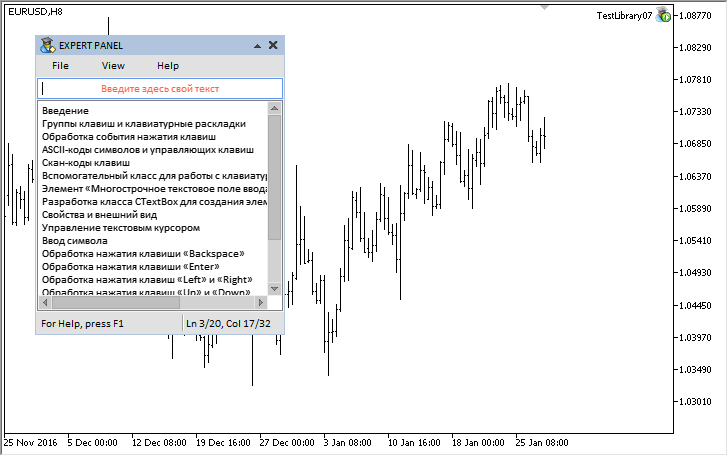Fig. 9. Graphical interface with demonstration of the Text box control

The test application featured in the article can be downloaded using the below link for further studying.

### Conclusion

Currently, the general schematic of the library for creating graphical interfaces looks as shown below: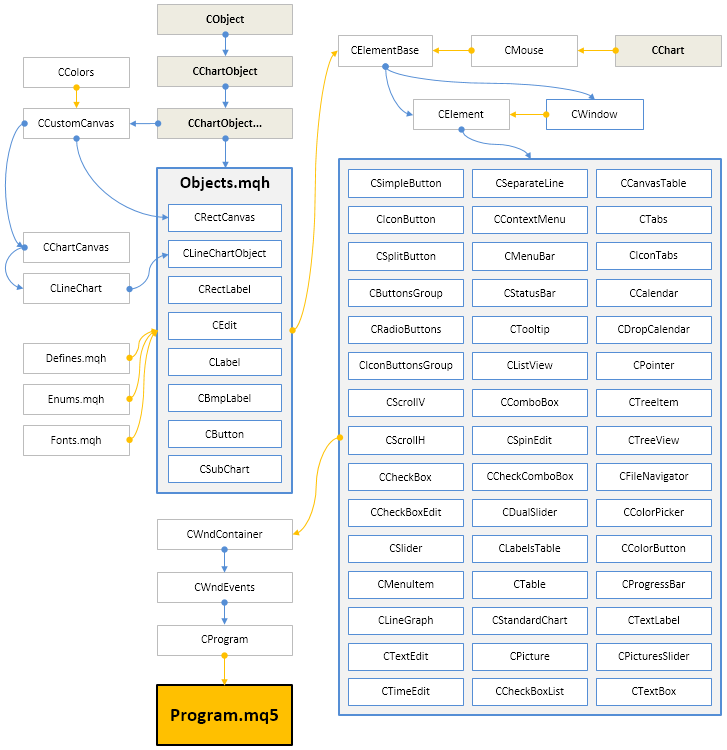Fig. 10. Structure of the library at the current stage of development.

The next version of the library will further develop and new functionality will be added to the already implemented controls. Below you can download the latest version of the library and files for testing.

If you have questions on using the material presented in those files, you can refer to the detailed description of the library development in one of the articles of this series or ask your question in the comments of this article.

Translated from Russian by MetaQuotes Software Corp.
Original article: https://www.mql5.com/ru/articles/3004

Attached files |

#### Other articles by this author

Last comments | Go to discussion (2)| 13 Mar 2017 at 05:24
MetaQuotes Software Corp.:

New article Graphical Interfaces X: The Multiline Text box control (build 8) has been published:

Author: Anatoli Kazharski

I've been messing with this for the past 3 days and there was a article.. So informative cut the time down dramatically thank you so much!!| 16 Mar 2017 at 11:01

Hello.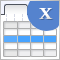Graphical interfaces X: New features for the Rendered table (build 9)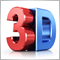3D Modeling in MQL5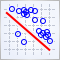Visualize this! MQL5 graphics library similar to 'plot' of R language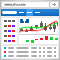Embed MetaTrader 4/5 WebTerminal on your website for free and make a profit